../
• 2020-01-04
• YOLO Neural Network Training With Darknet
Also see my recent posts on how to train an artificial neural network, and how to use a neural network from within a C++ application.

## Summary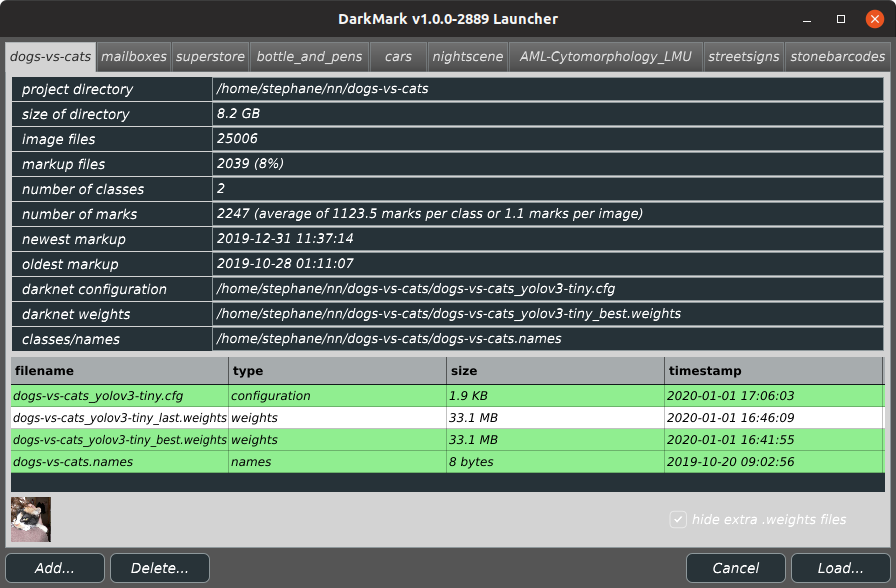Spent quite a bit of time running numerous tests to see how different parameters impact the result of training YOLO artificial neural networks using the Darknet framework. This is the network I was using:

The two variables I was working with is the ratio of training/validation files, and the max_batches variable in the YOLO .cfg file (with the other associated values when you change max_batches).

With the large number of marked up images, I varied the percentage of training/validation between 50%, 70%, 85%, and 99%.

I then modified max_batches between 1K, 2K, 5K, 10K, 20K, and finally 40K.

At the low end, training on a GeForce RTX 2070 with max_batches set to 1000 took 5 minutes. At the top end, max_batches set to 40000 took nearly 4 hours.

(I was curious and also ran a CPU test. On a 3.2 GHz 16-core desktop computer, I tried to train without the use of a GPU. It took 5m 39s to complete 10 iterations. At this rate, training with max_batches set to 40K would take approximately 377 hours, or nearly 16 days.)

## Results

The original image I used to test the ANN contains 2 dogs and is not marked up in this project, thus it never shows up in either the training or validation set: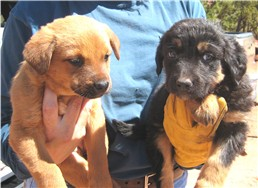At the low end of max_batches, the training/validation ratio can have a significant impact on the results, making it seem as if the ANN is nearly (prematurely?) usable. For example, see the results of max_batches=2000 and training=85%: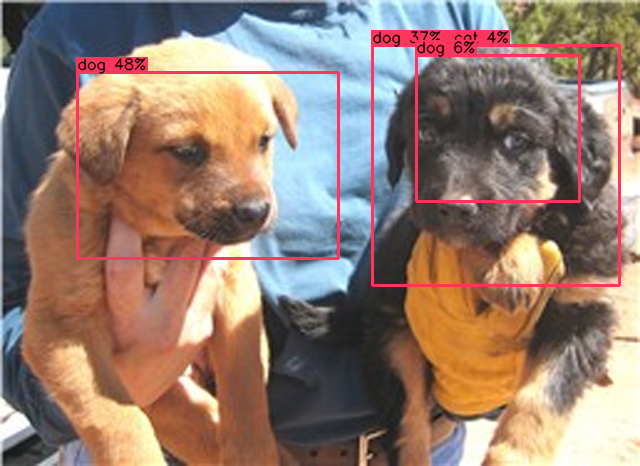However, attempting to apply this ANN to other images shows this is not a usable network: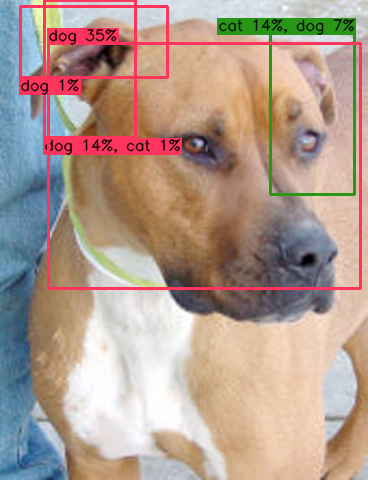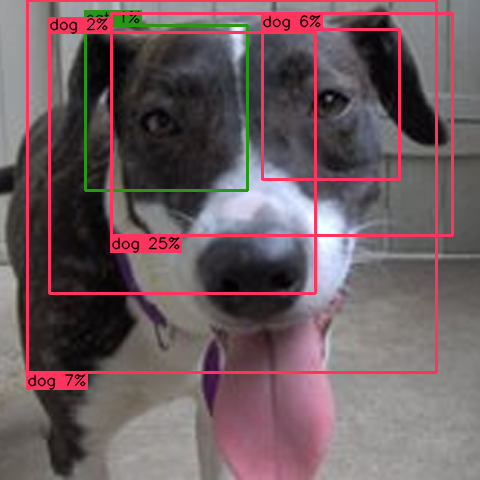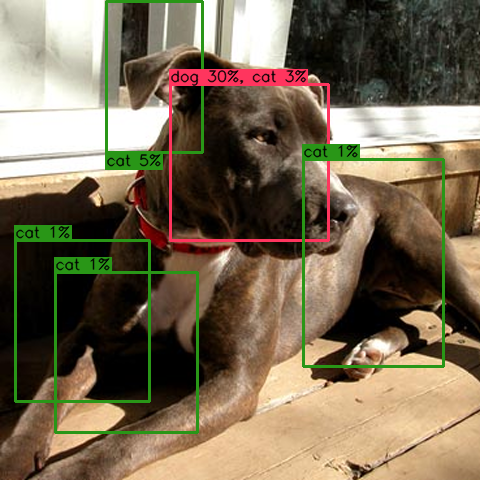Once max_batches is significantly increased, the results become mouch more reliable, and the training/validation ratio has less impact on the results.

Those same images after training with max_batches set to 40K instead of 2K: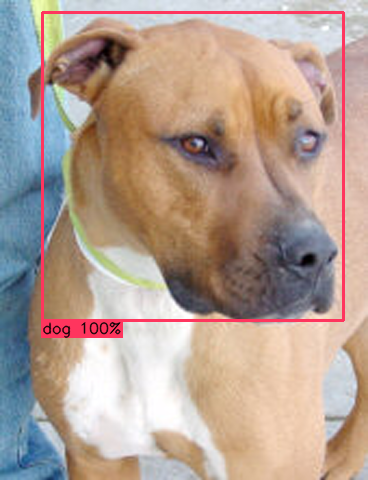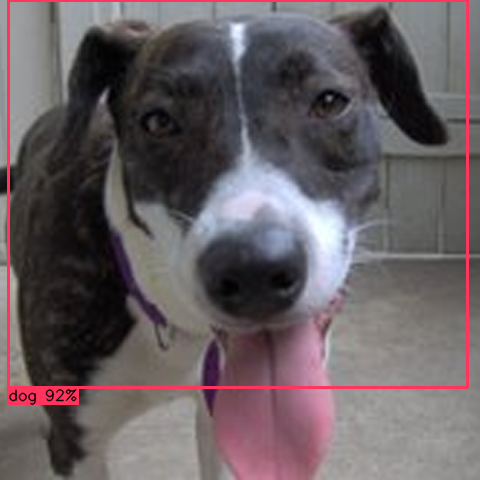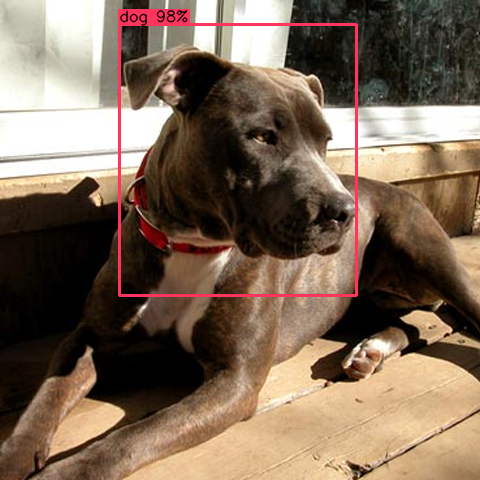## Details

This is what the Yolov3-tiny artificial neural network looked like with the various combinations of training percentage and max_batches:

50% training, 50% validation 70% training, 30% validation 85% training, 15% validation 99% training, 1% validation
max_batches=1000
time=5m 25s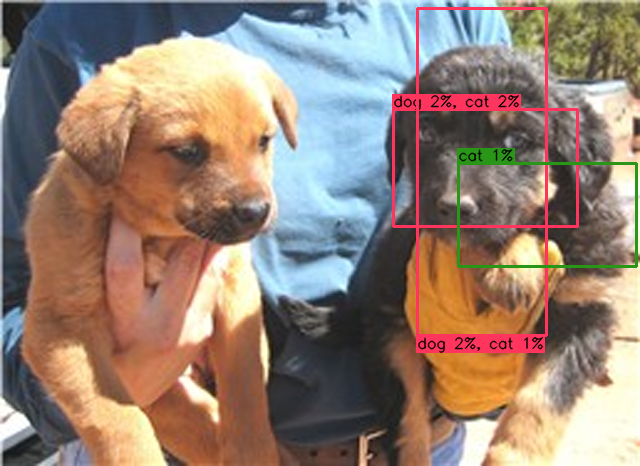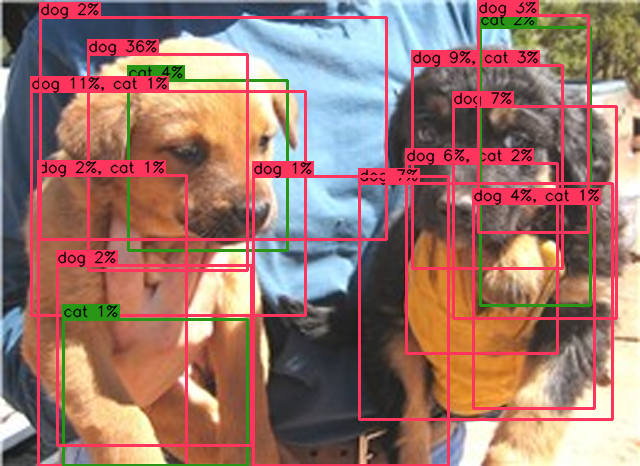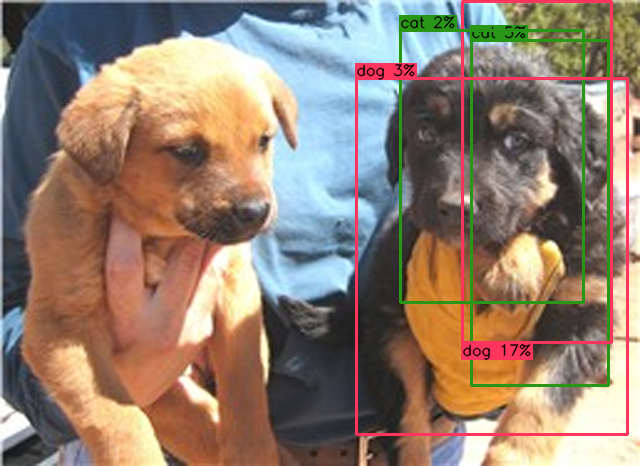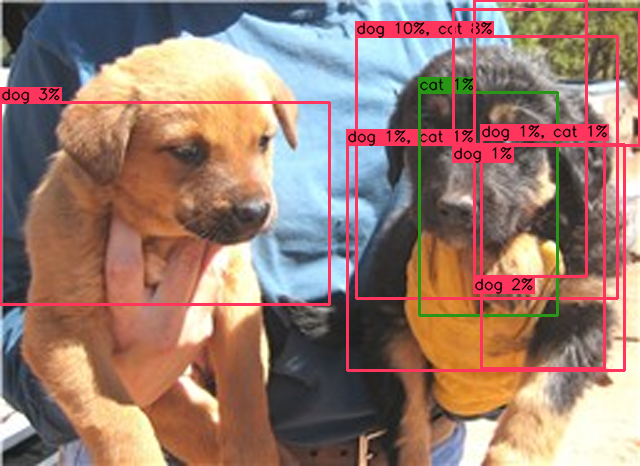max_batches=2000
time=11m 4s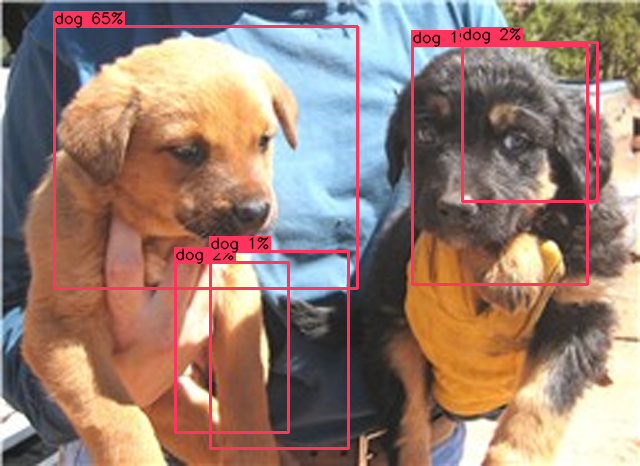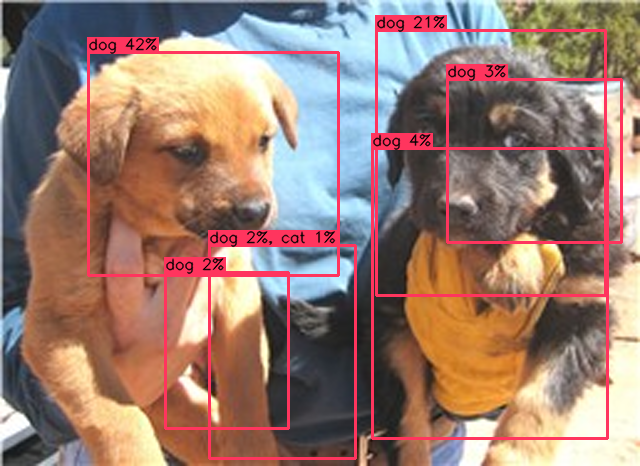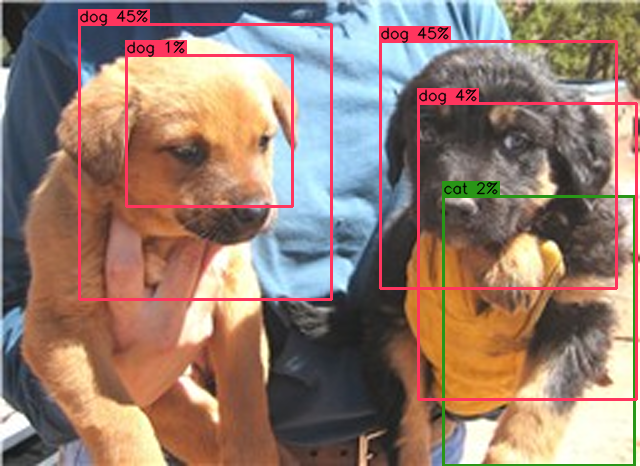max_batches=5000
time=29m 13s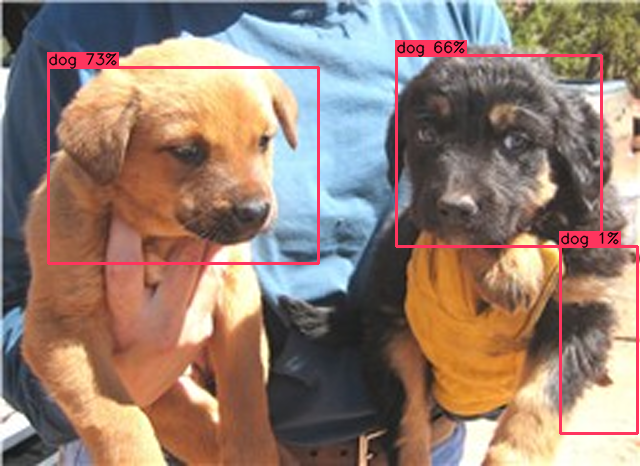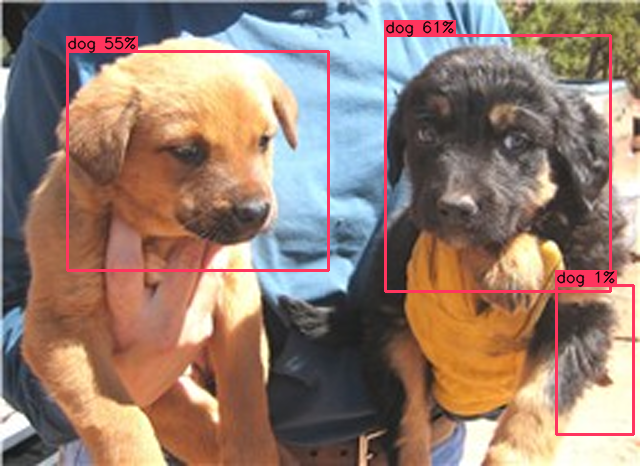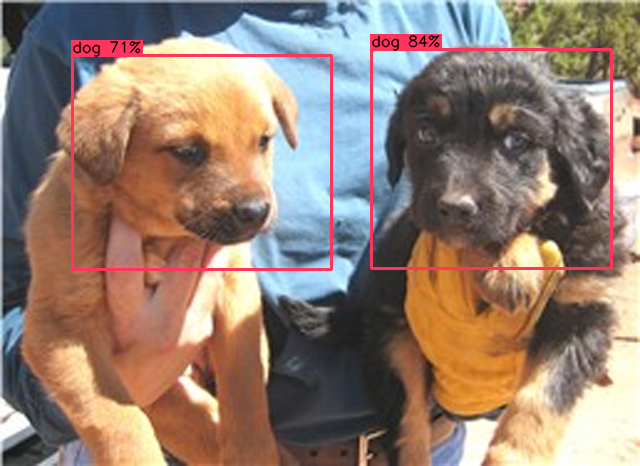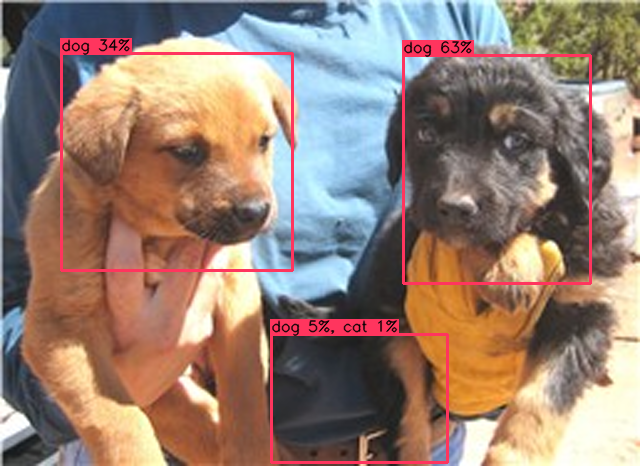max_batches=10000
time=57m 22s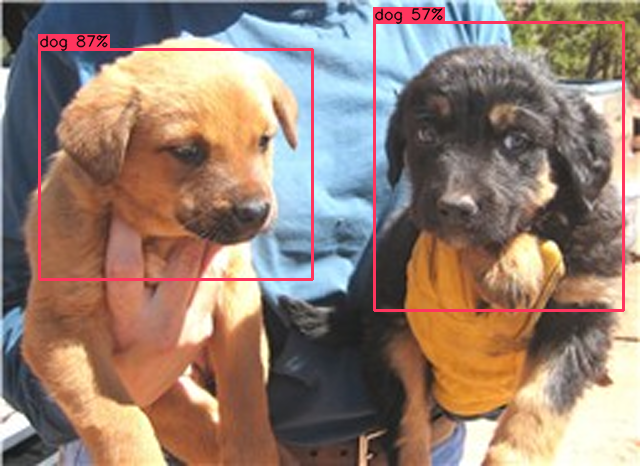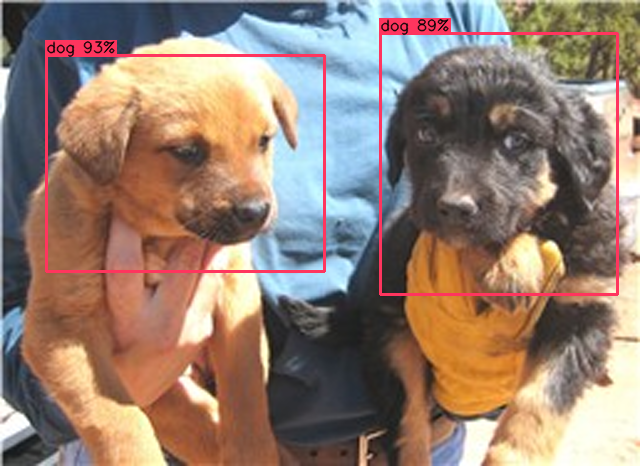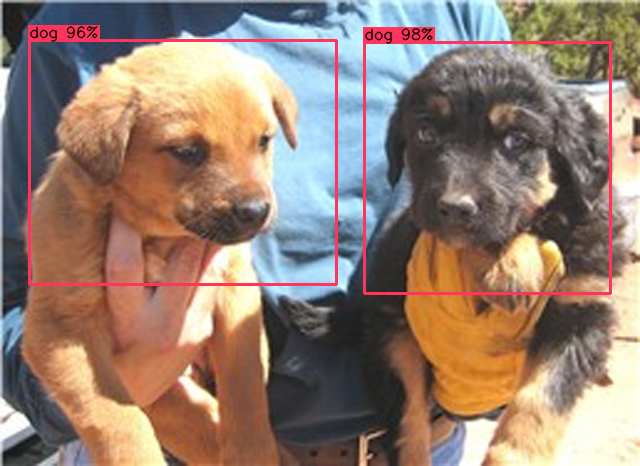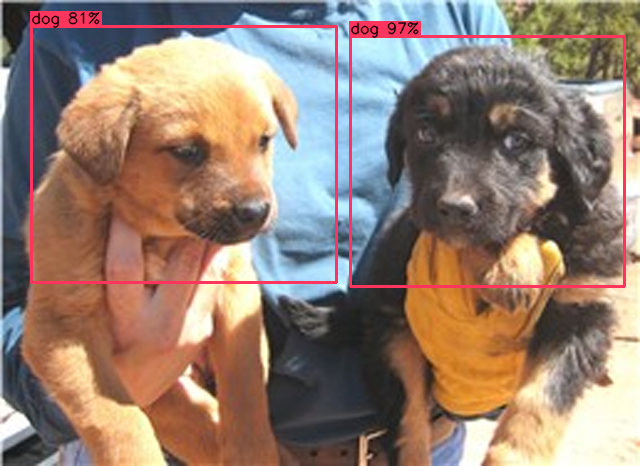max_batches=20000
time=1h 54m 30s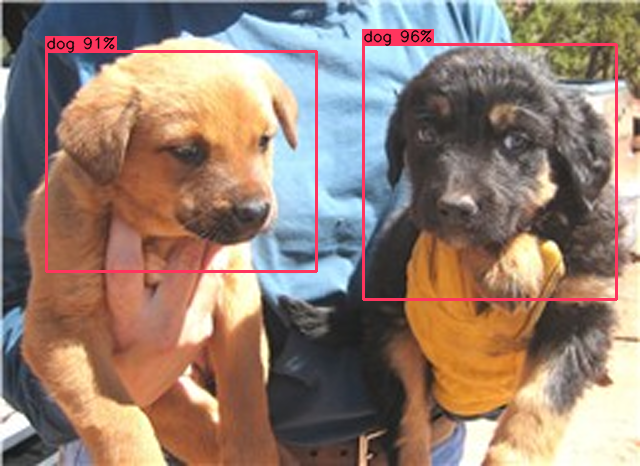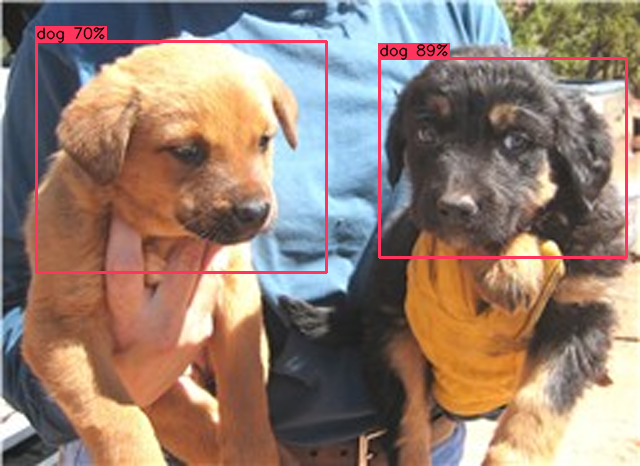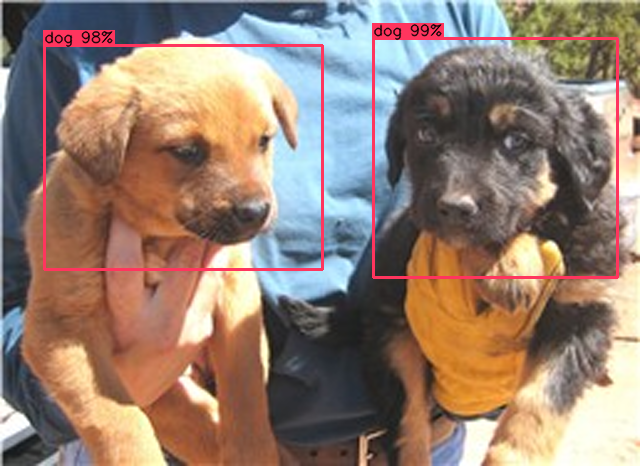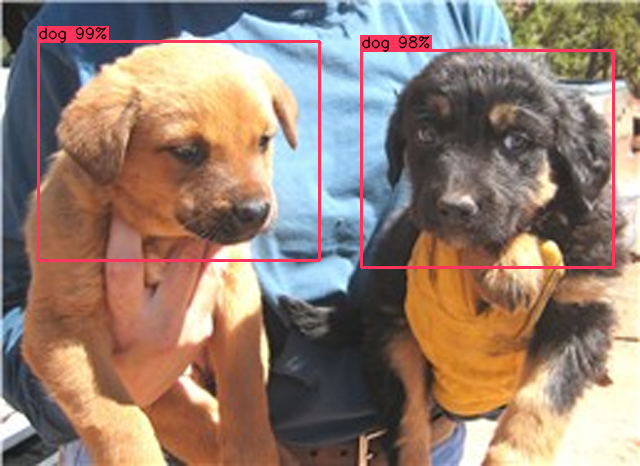max_batches=40000
time=3h 48m 12s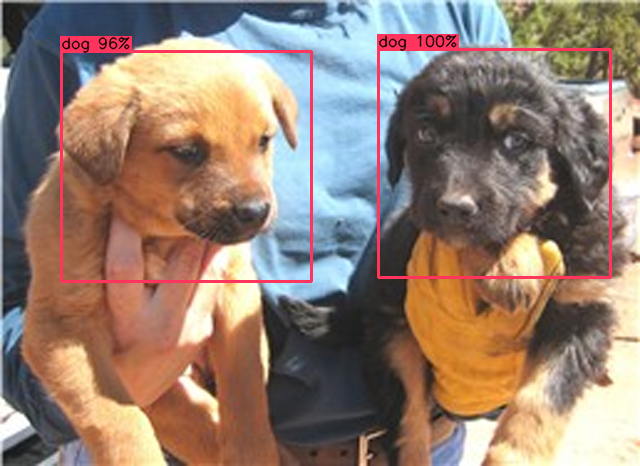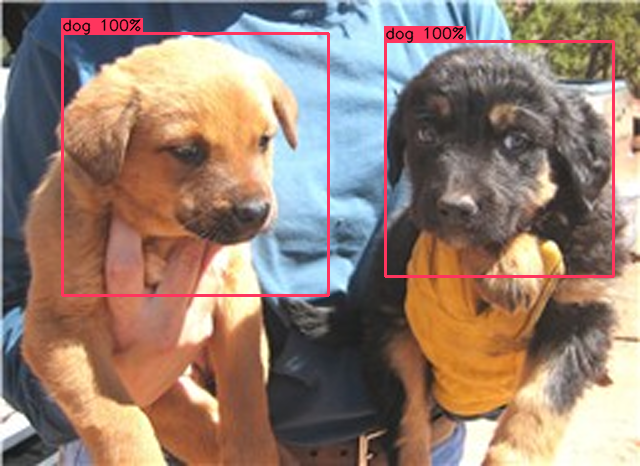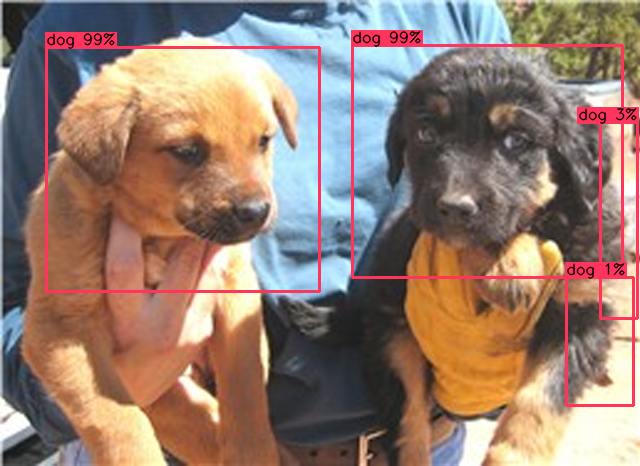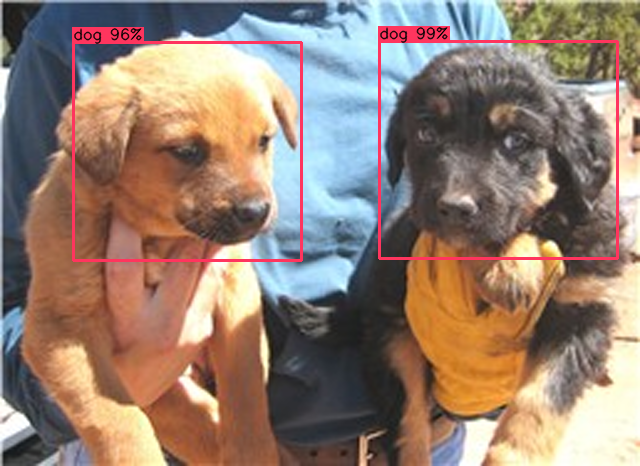## Charts

I recorded the loss and mAP (mean average precision) chart output by Darknet during training for each variation of the artificial neural network. There were no surprises, which matches the consistent results from the numerous test images in the table above.

50% training 70% training 85% training 99% training
max_batches=1000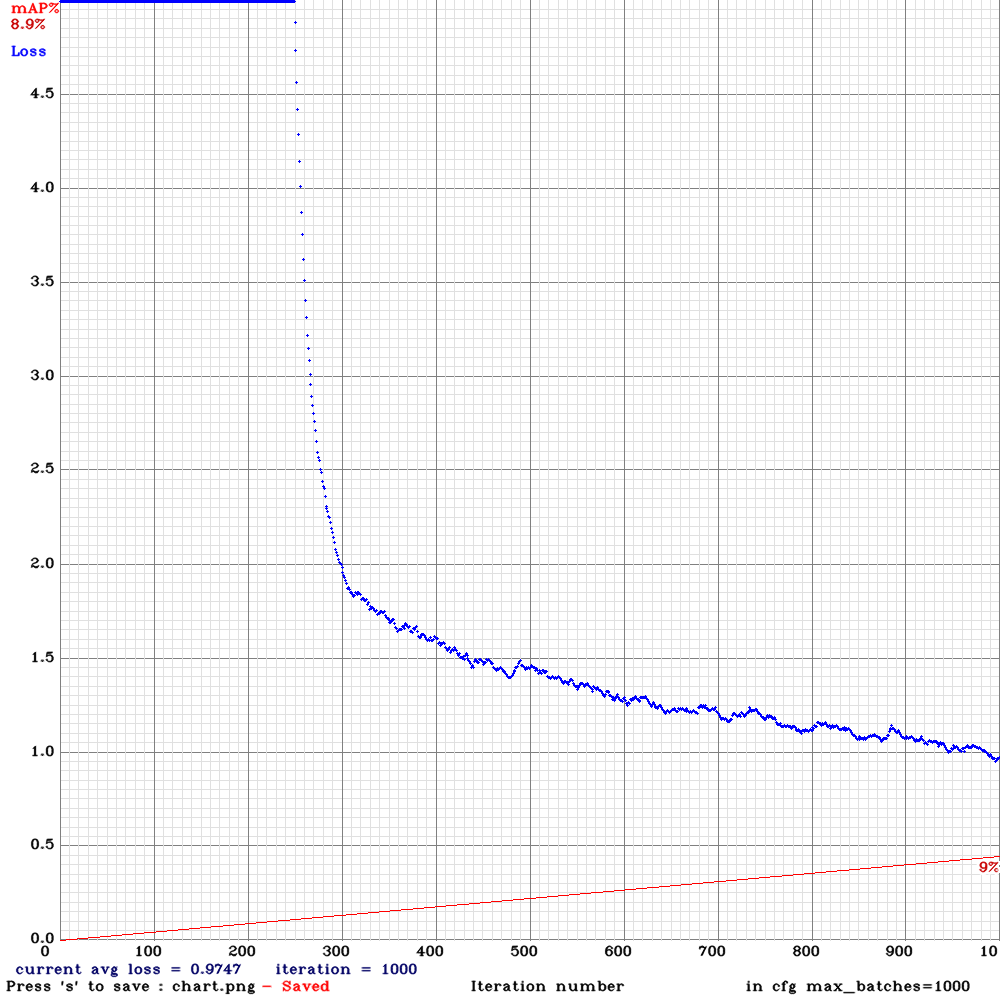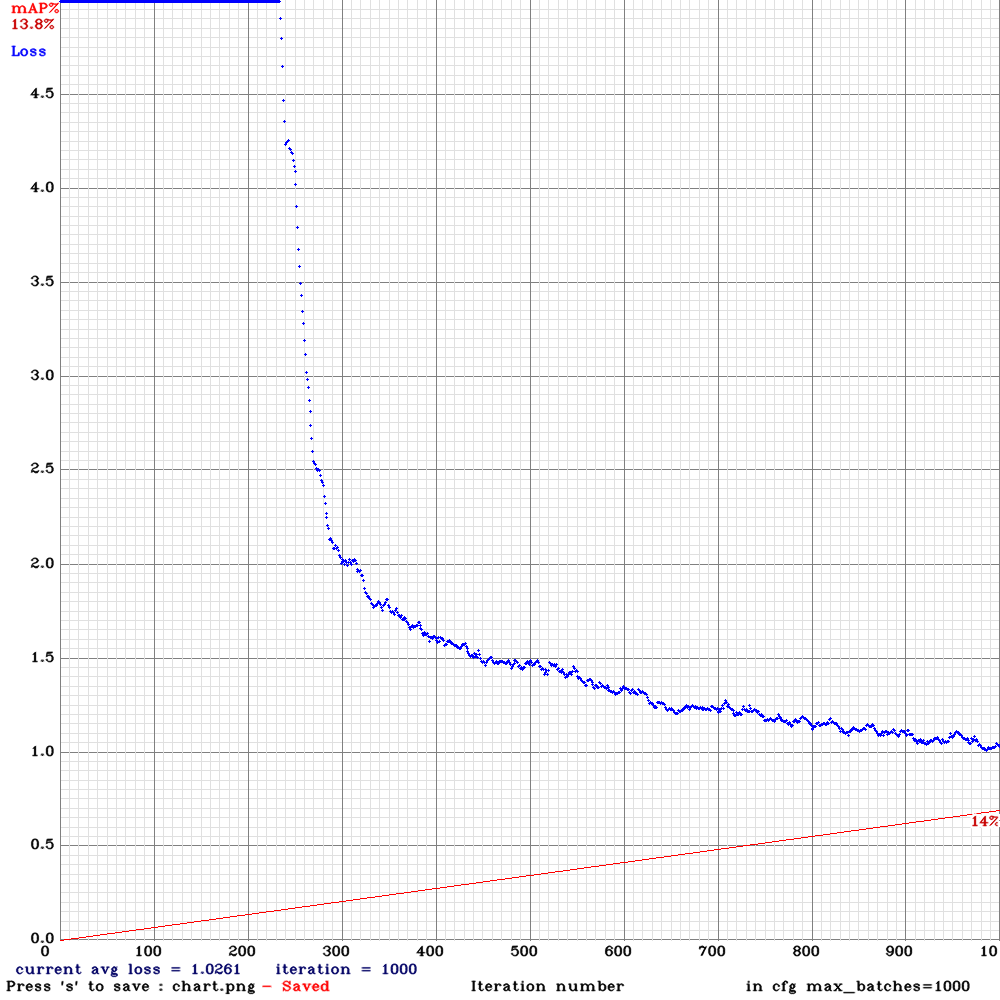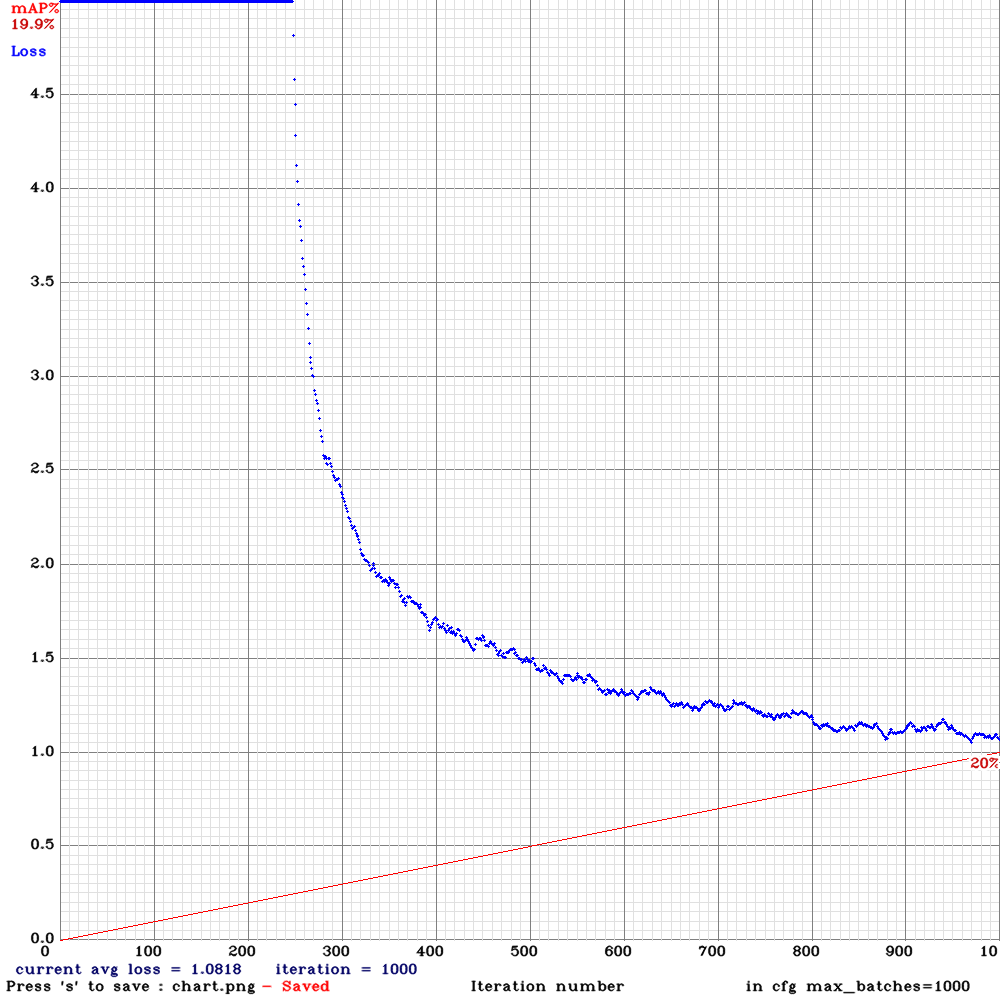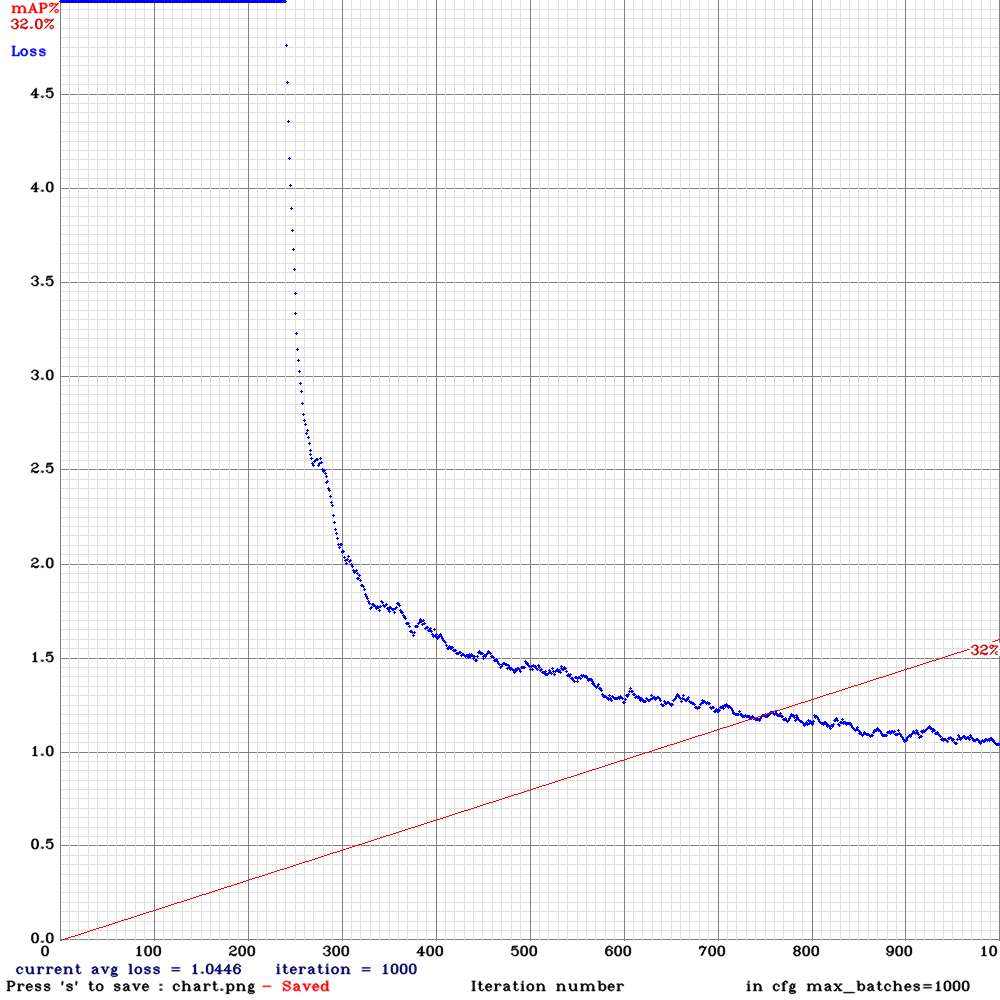max_batches=2000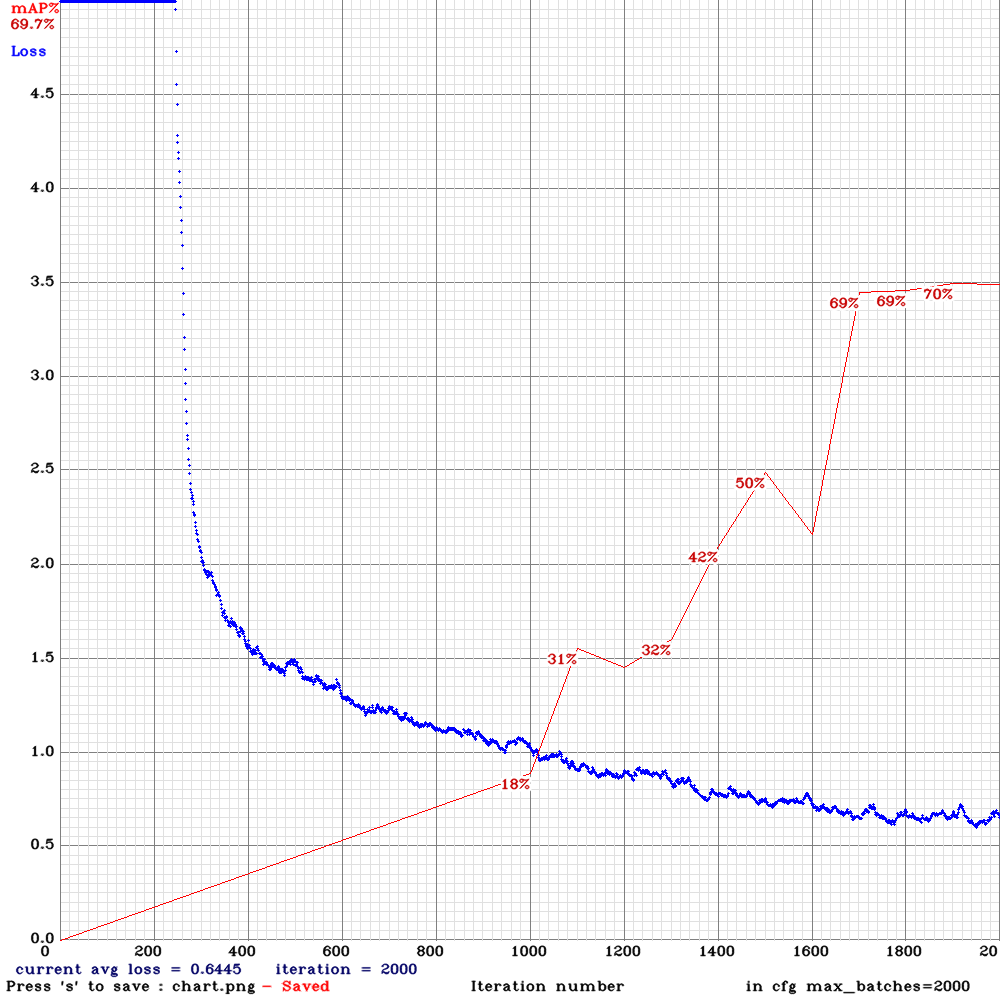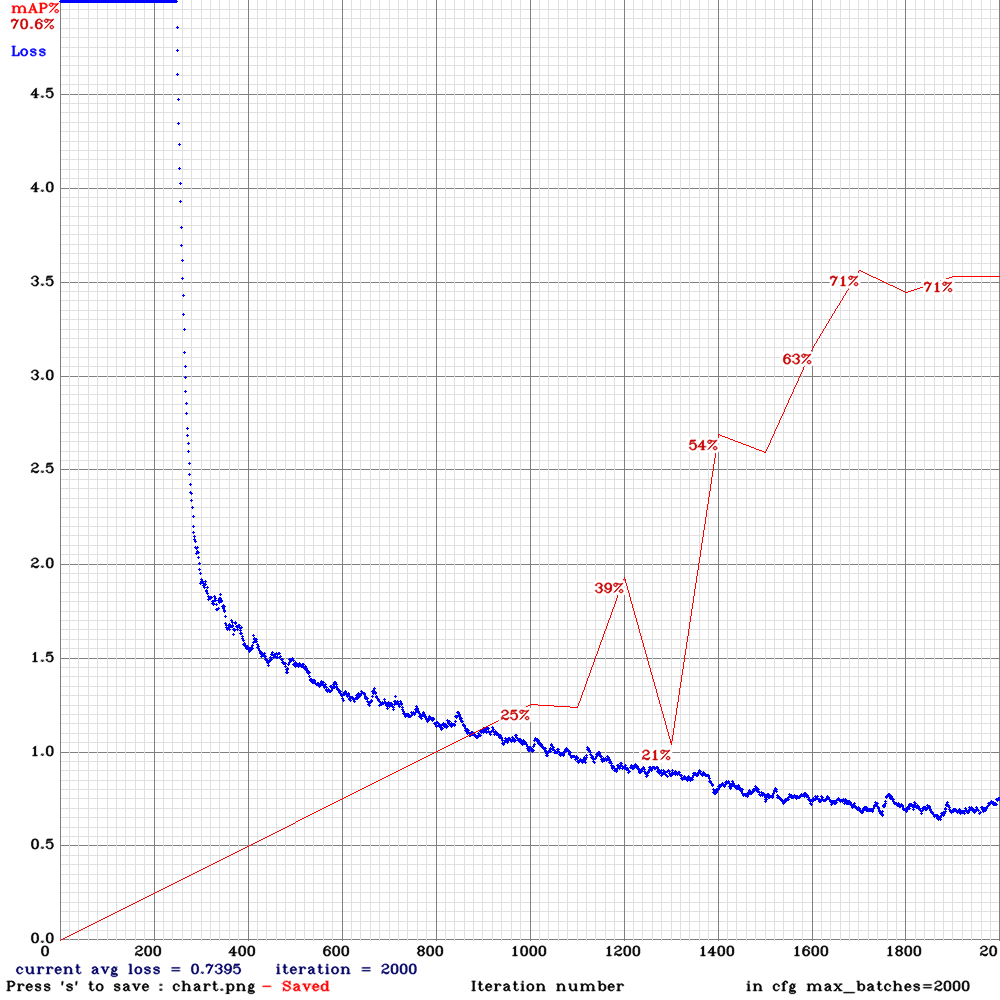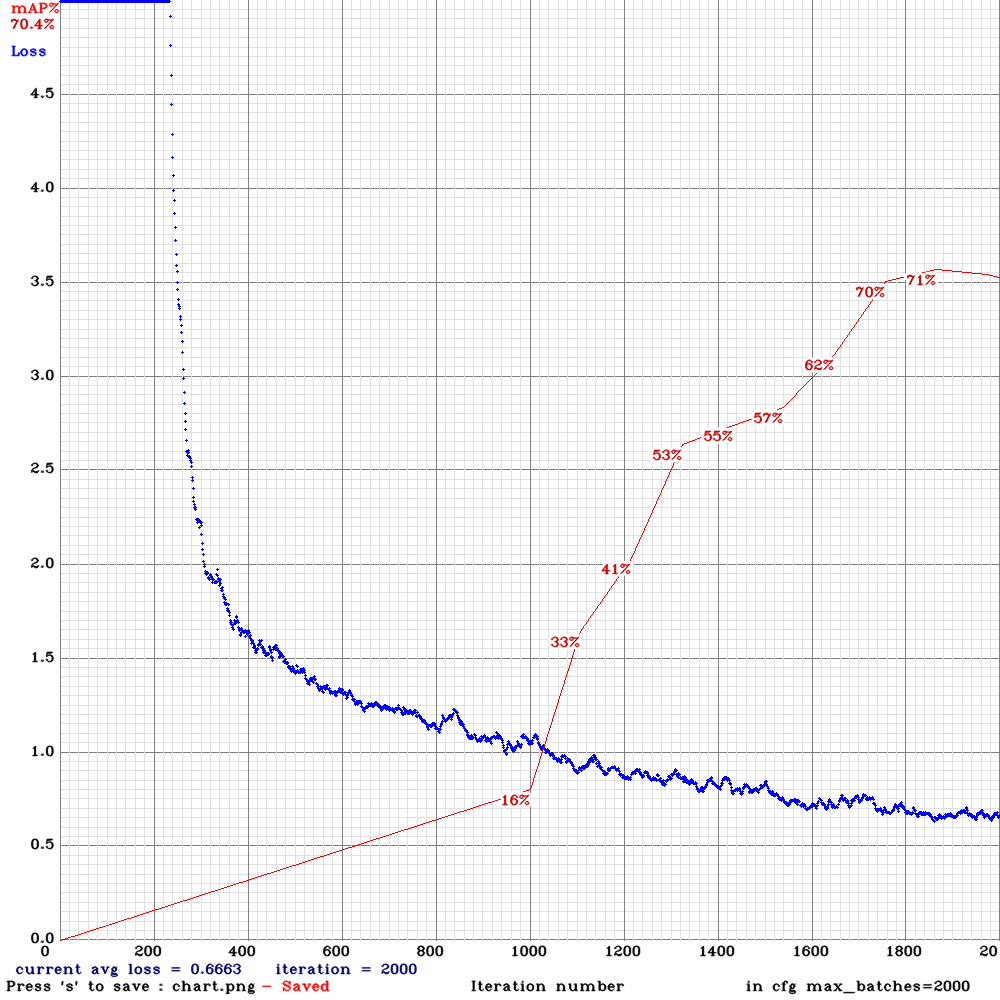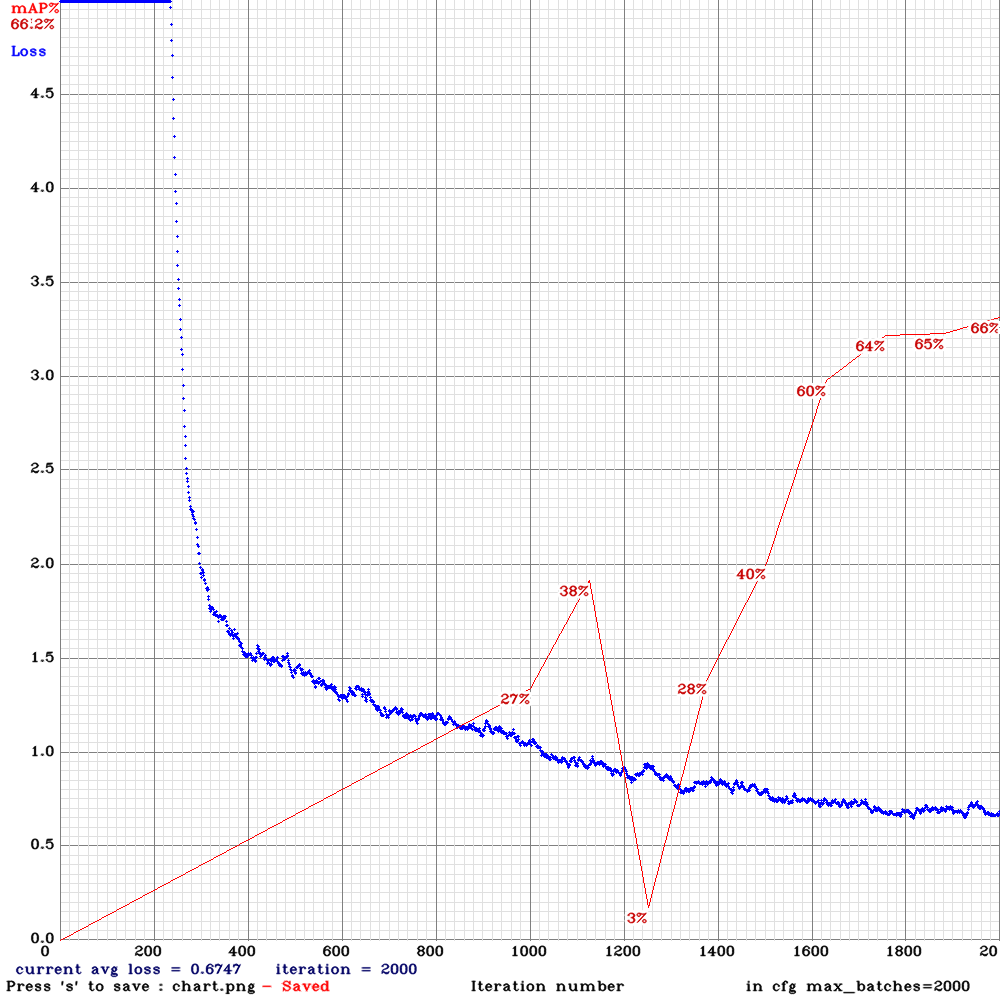max_batches=5000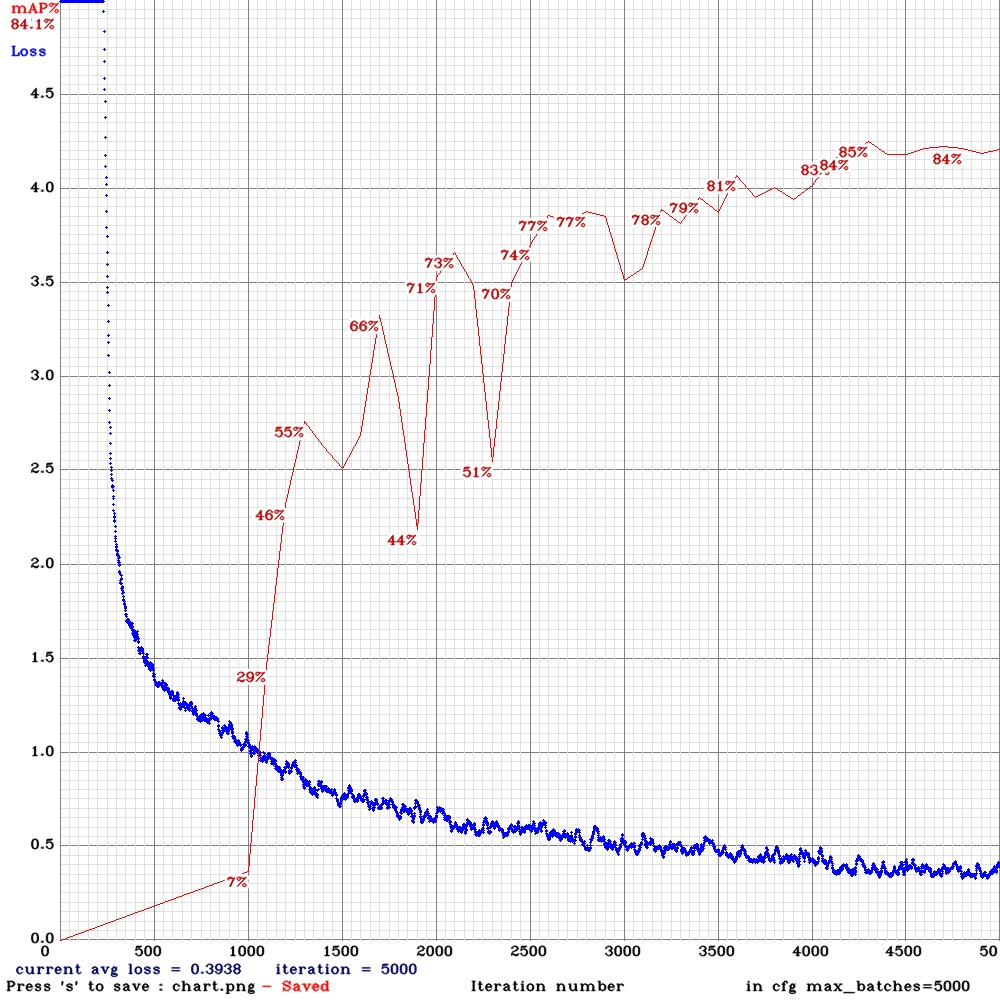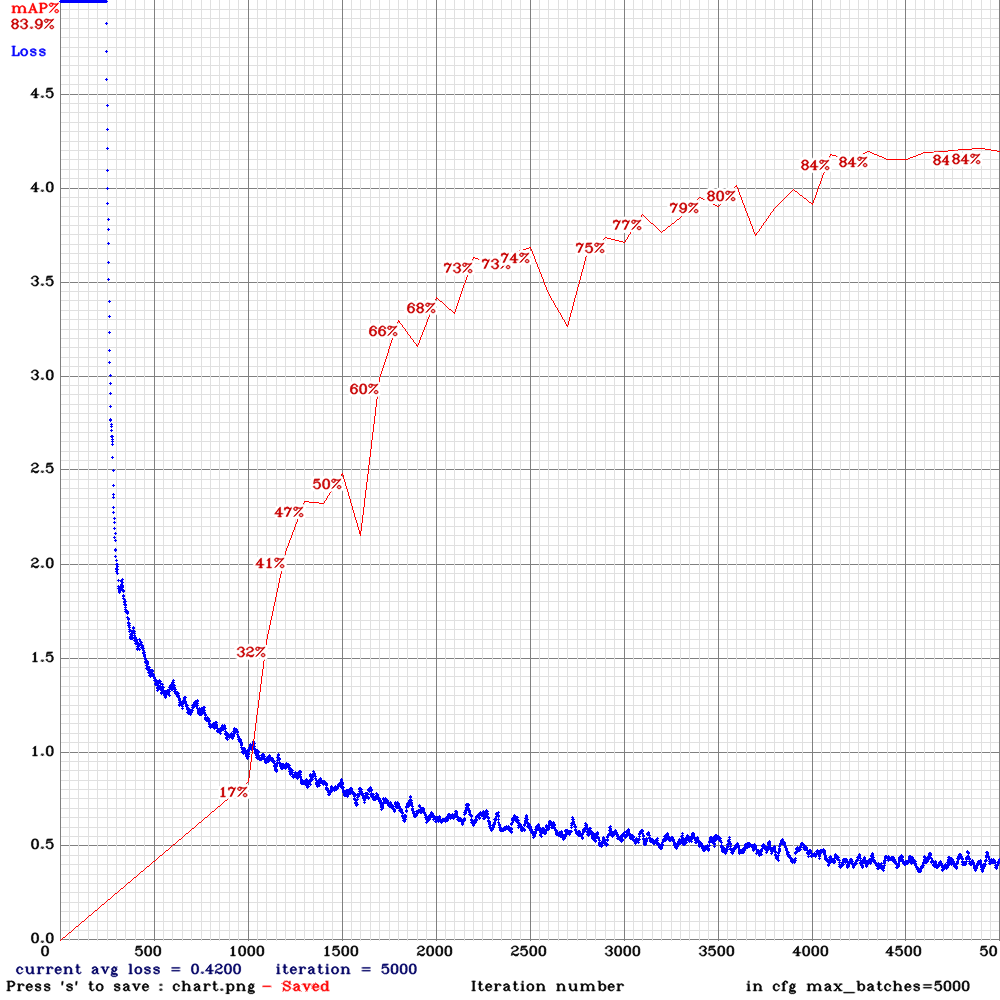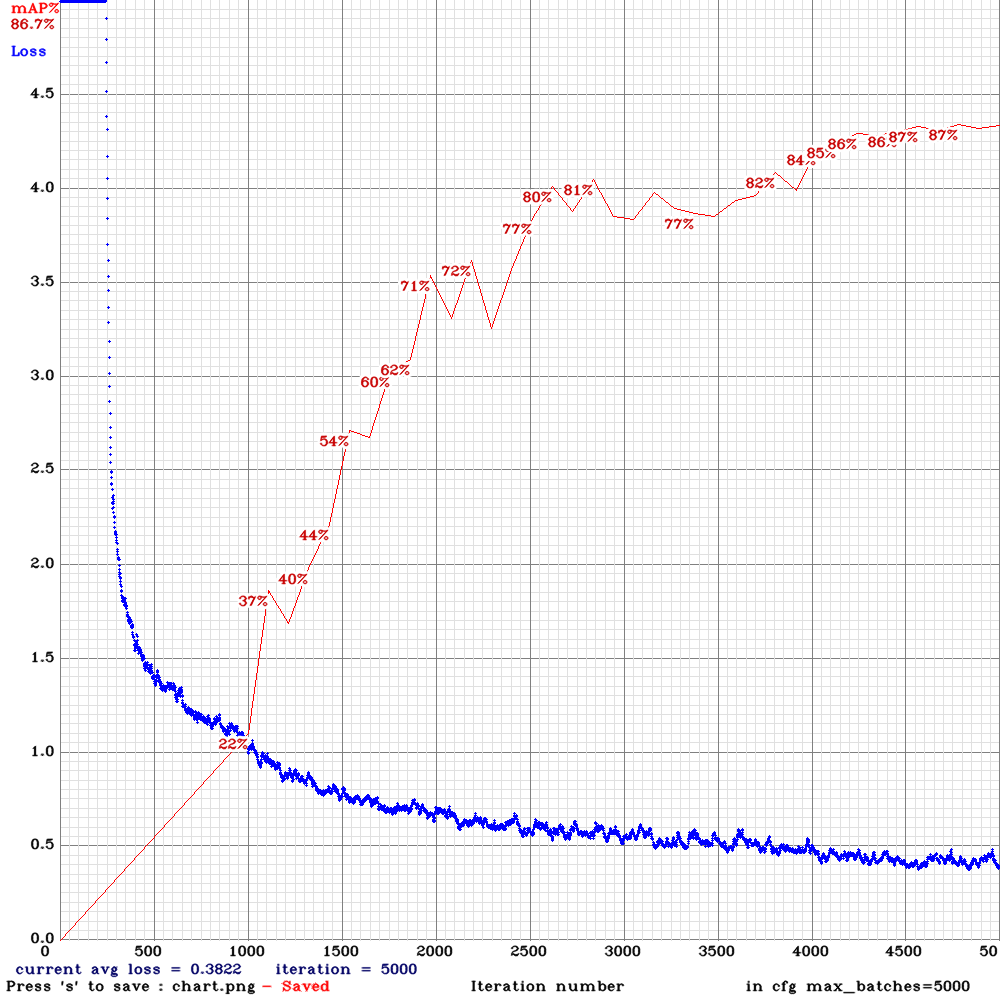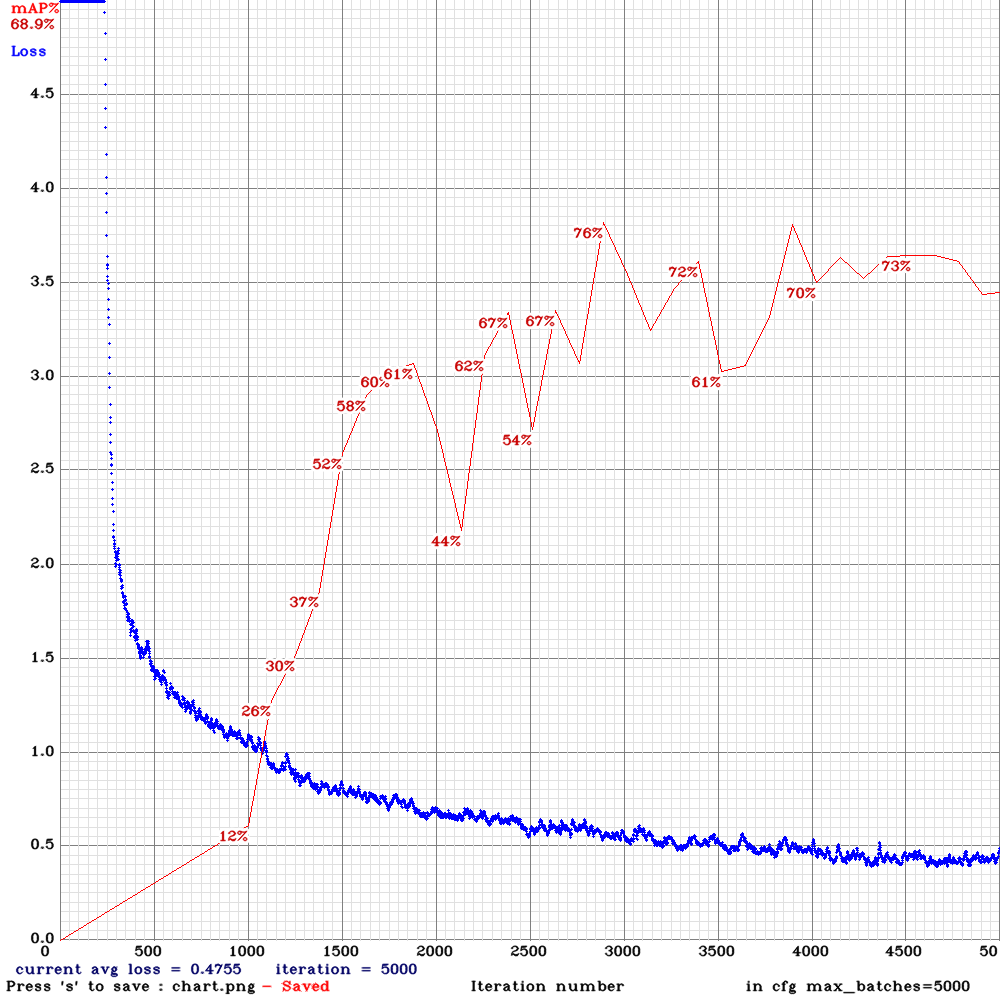max_batches=10000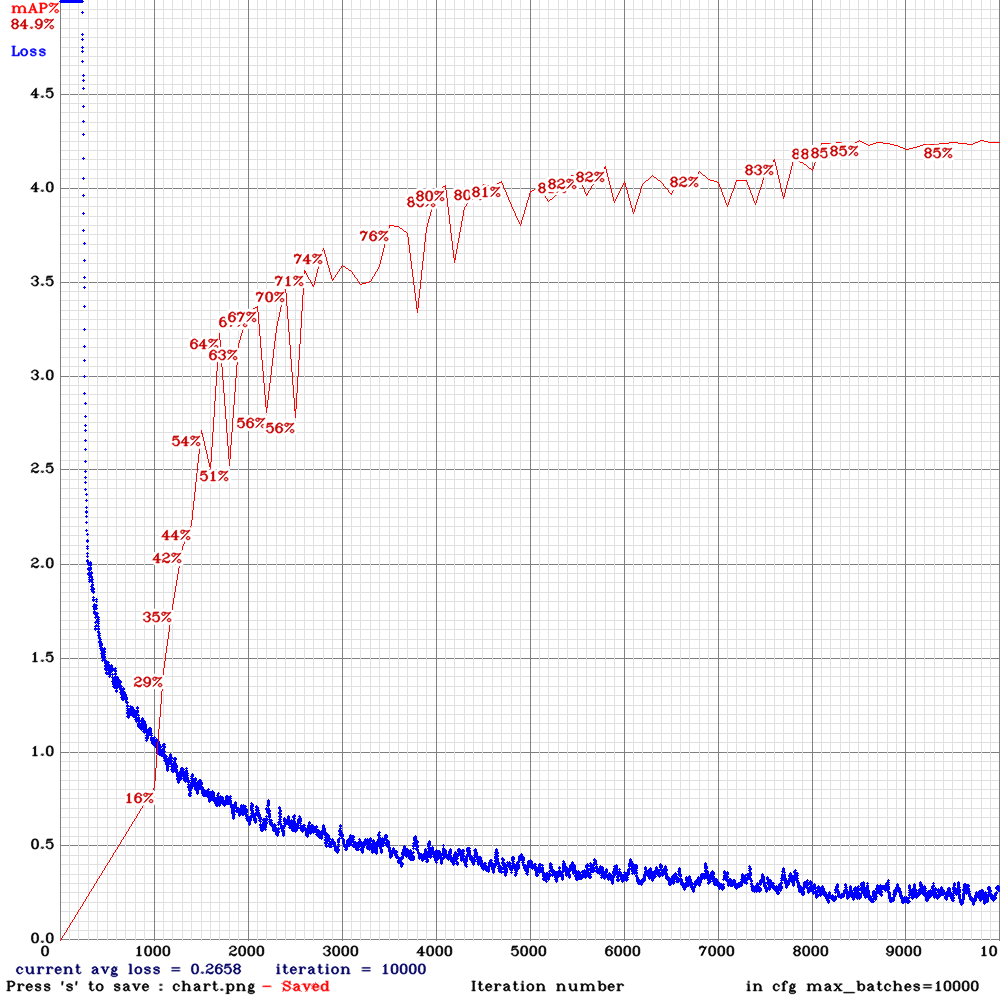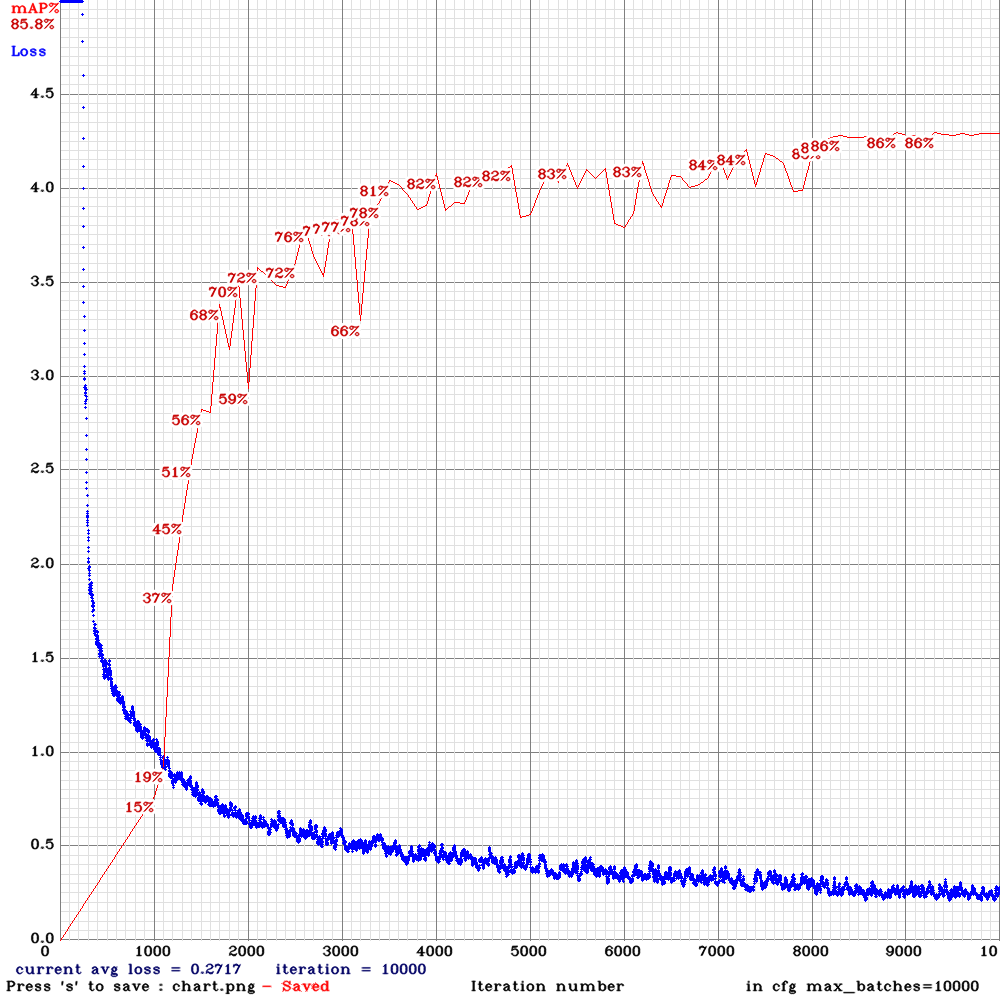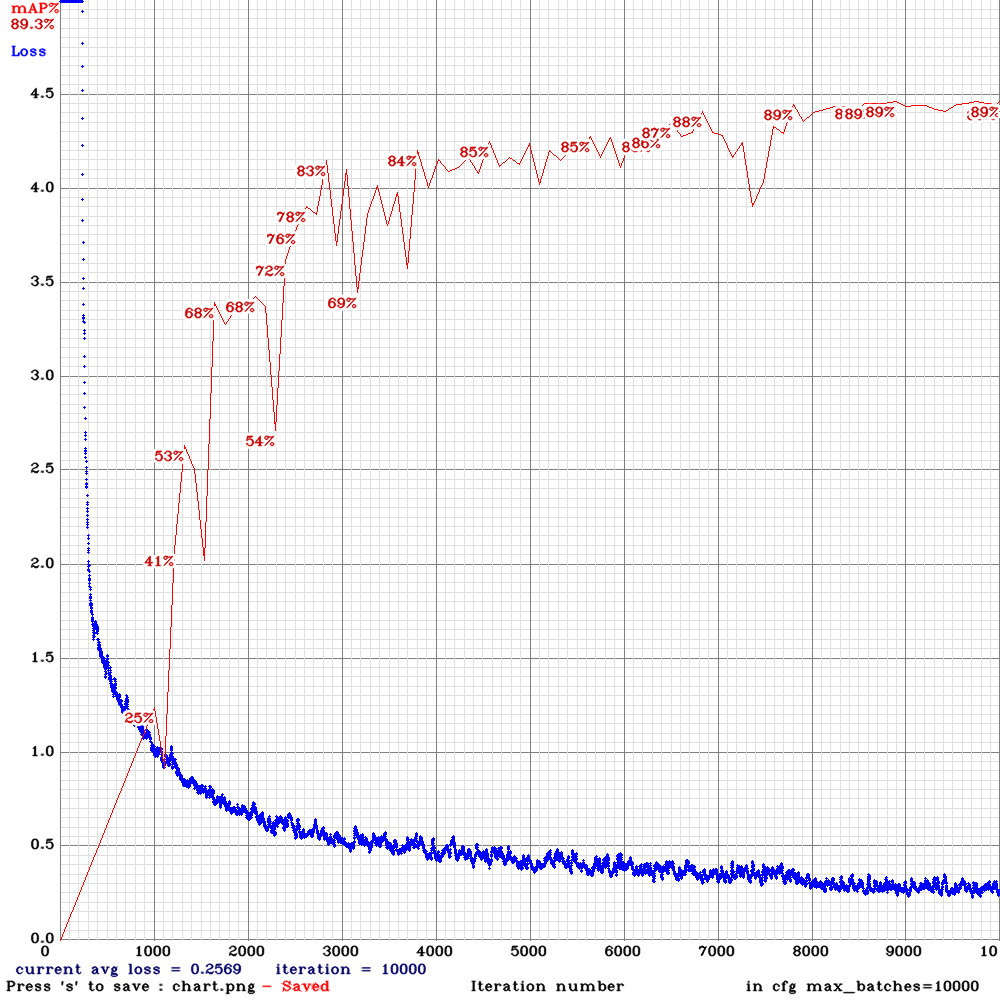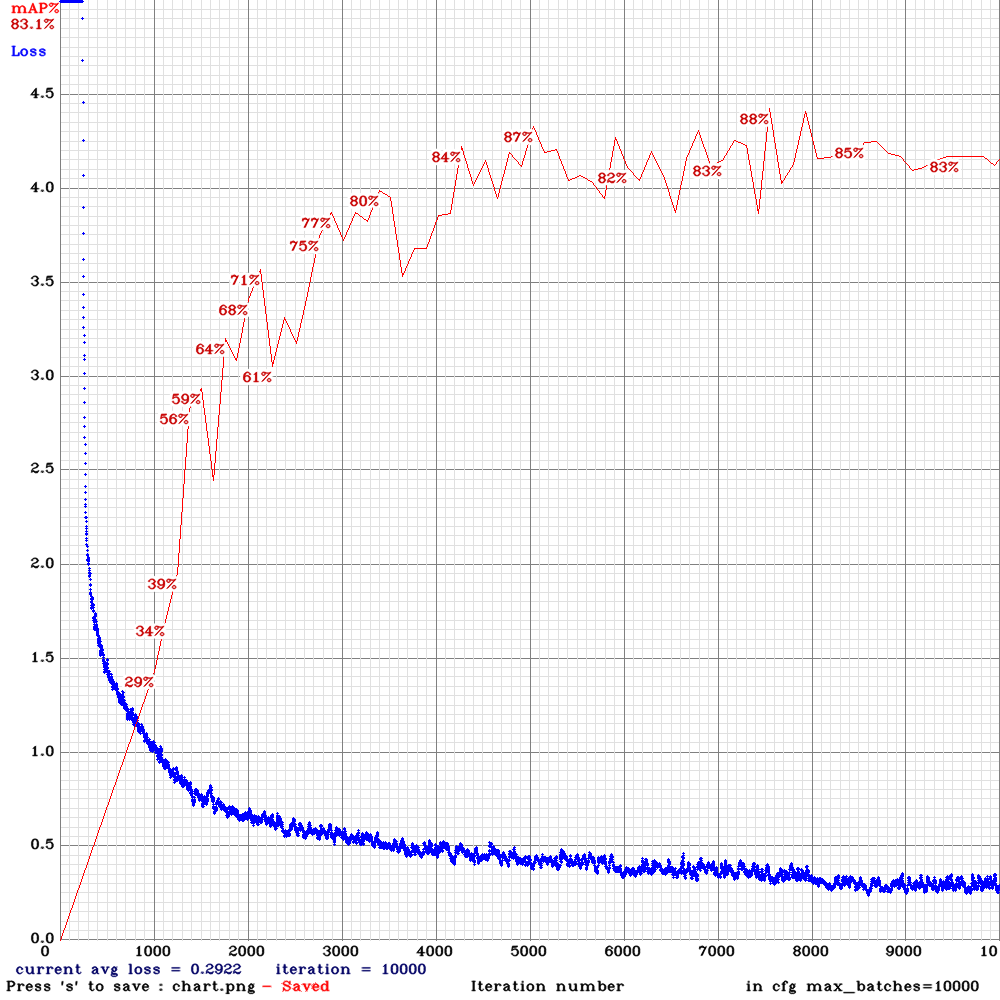max_batches=20000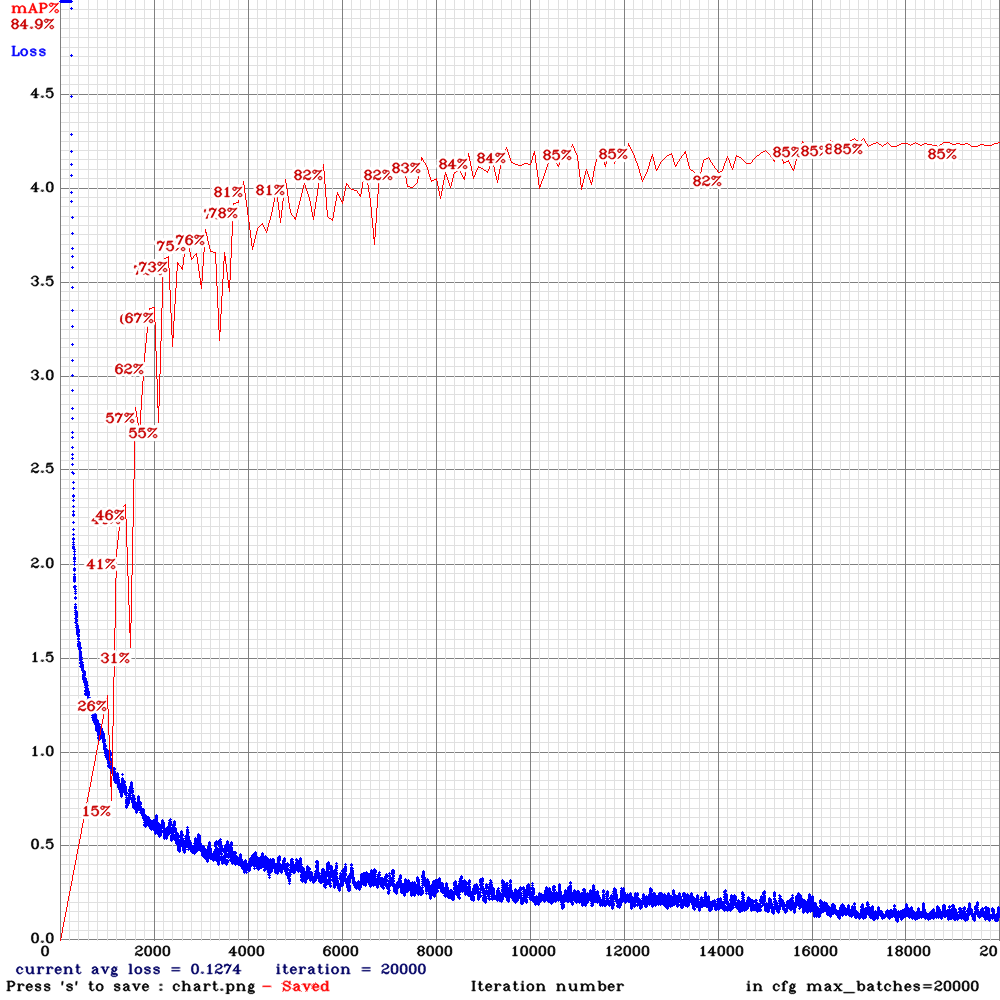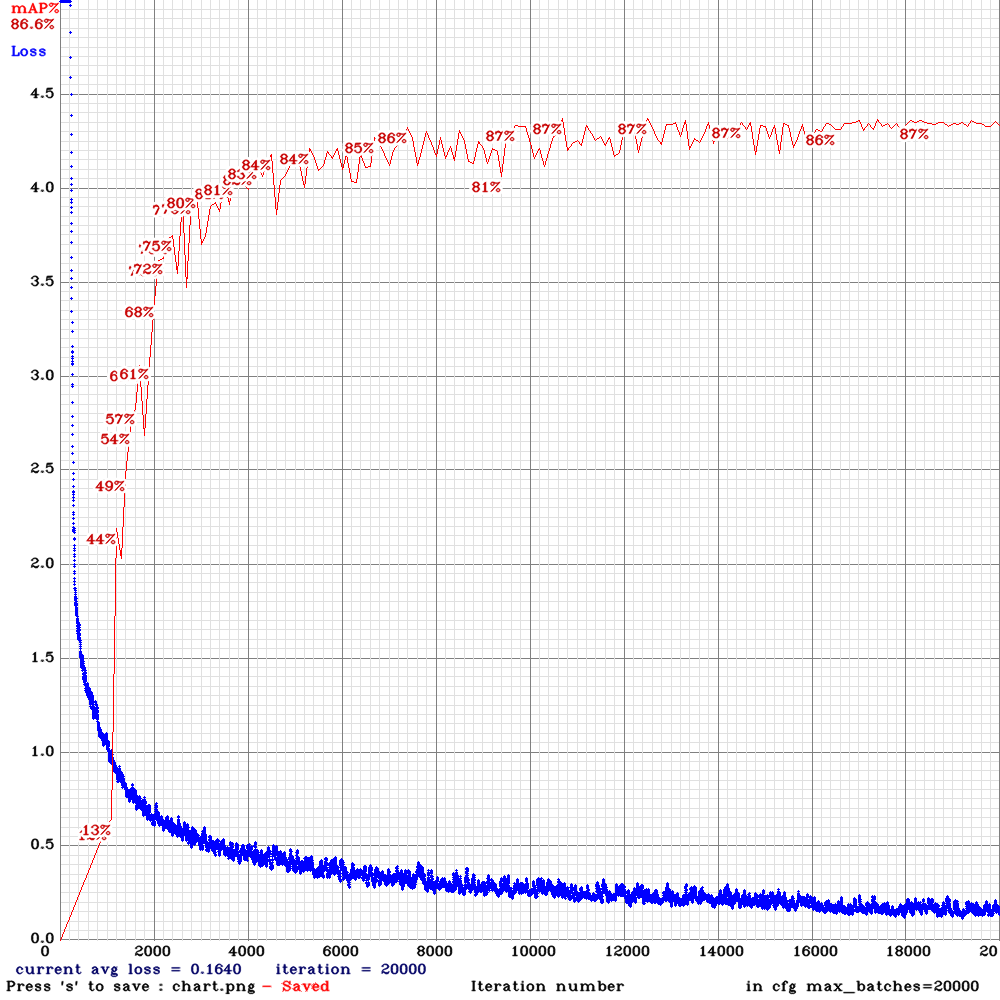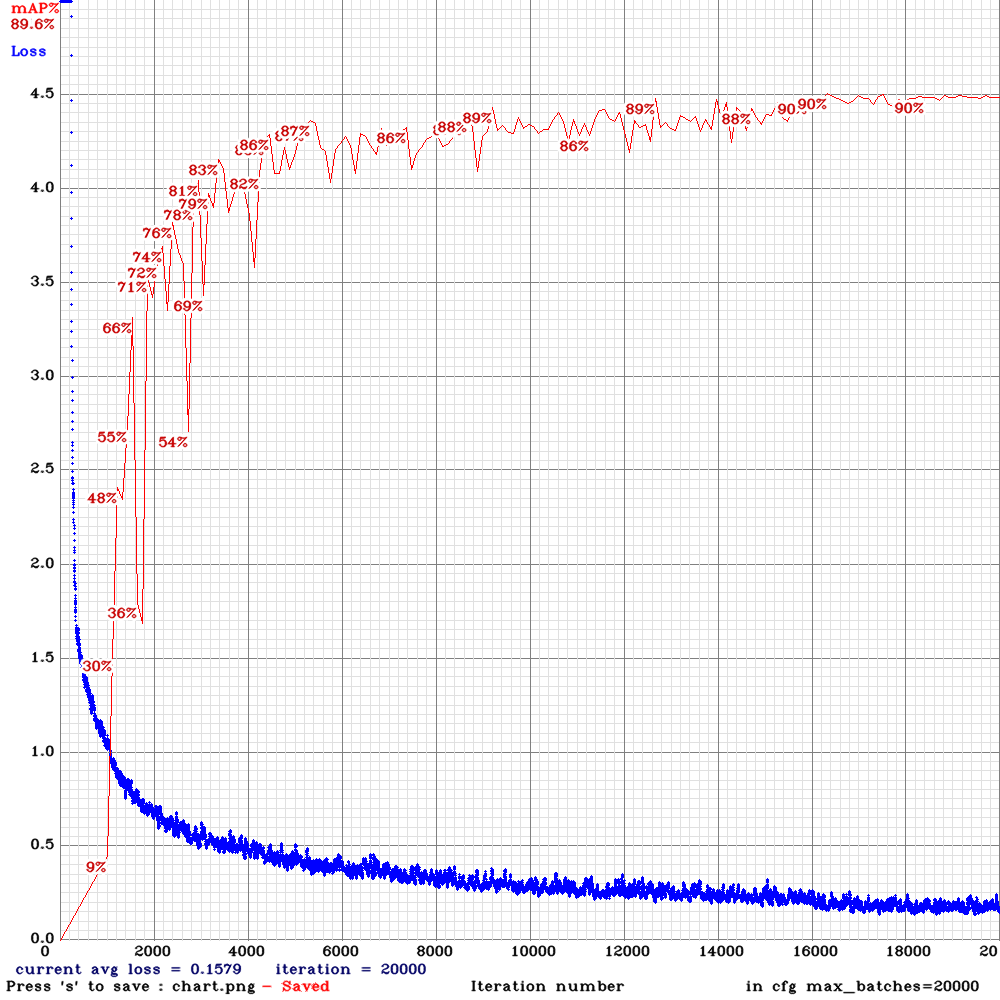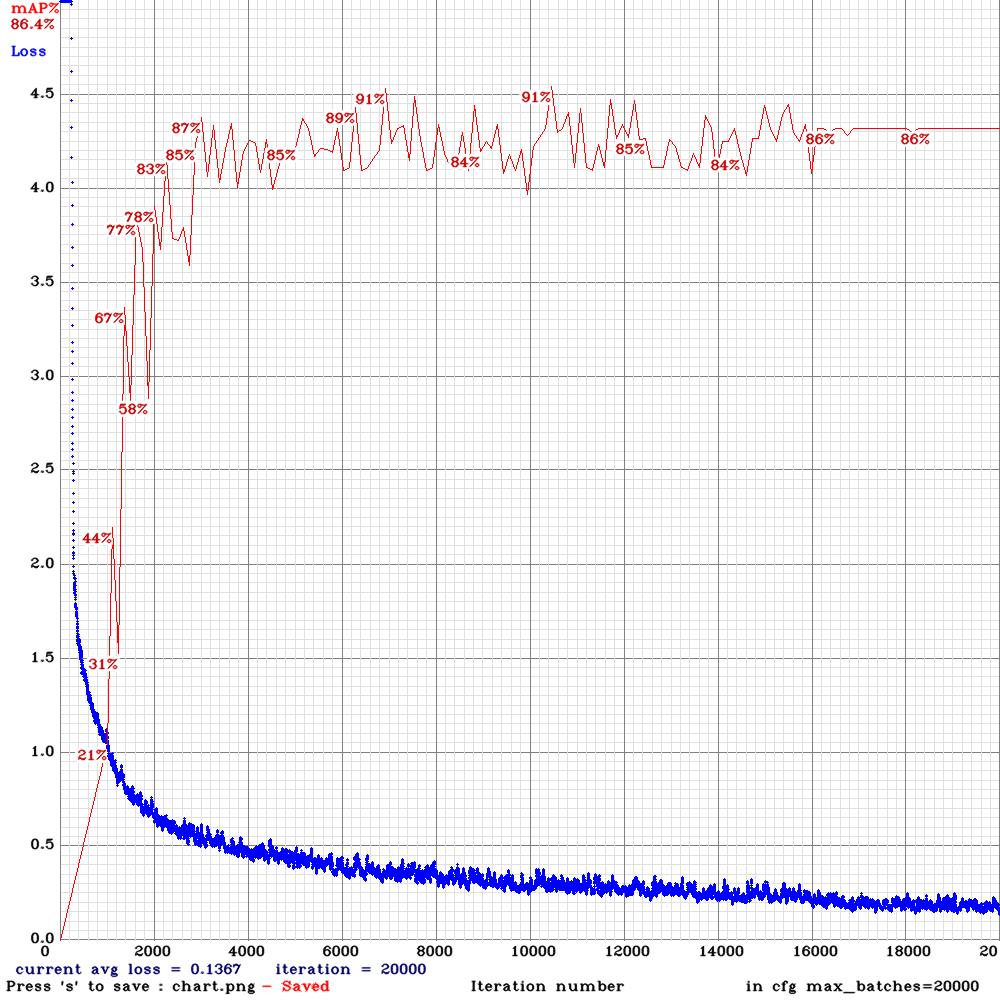max_batches=40000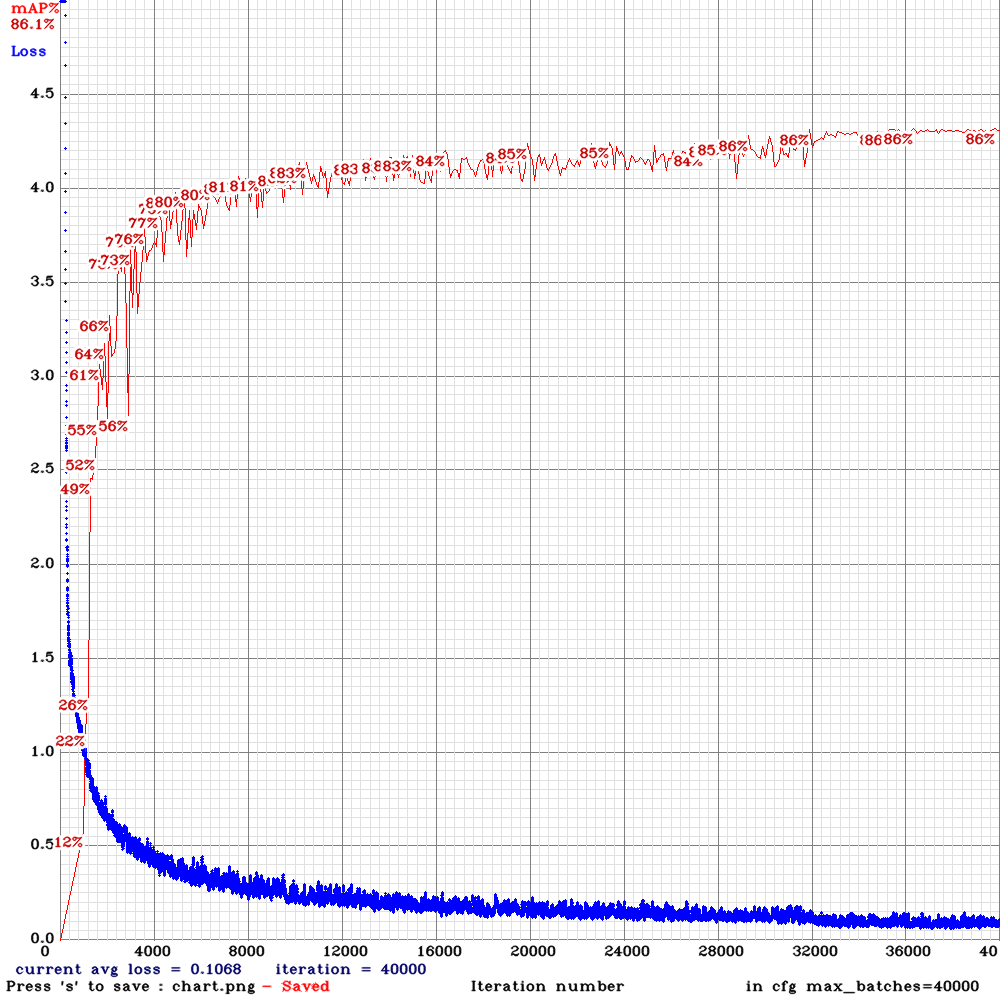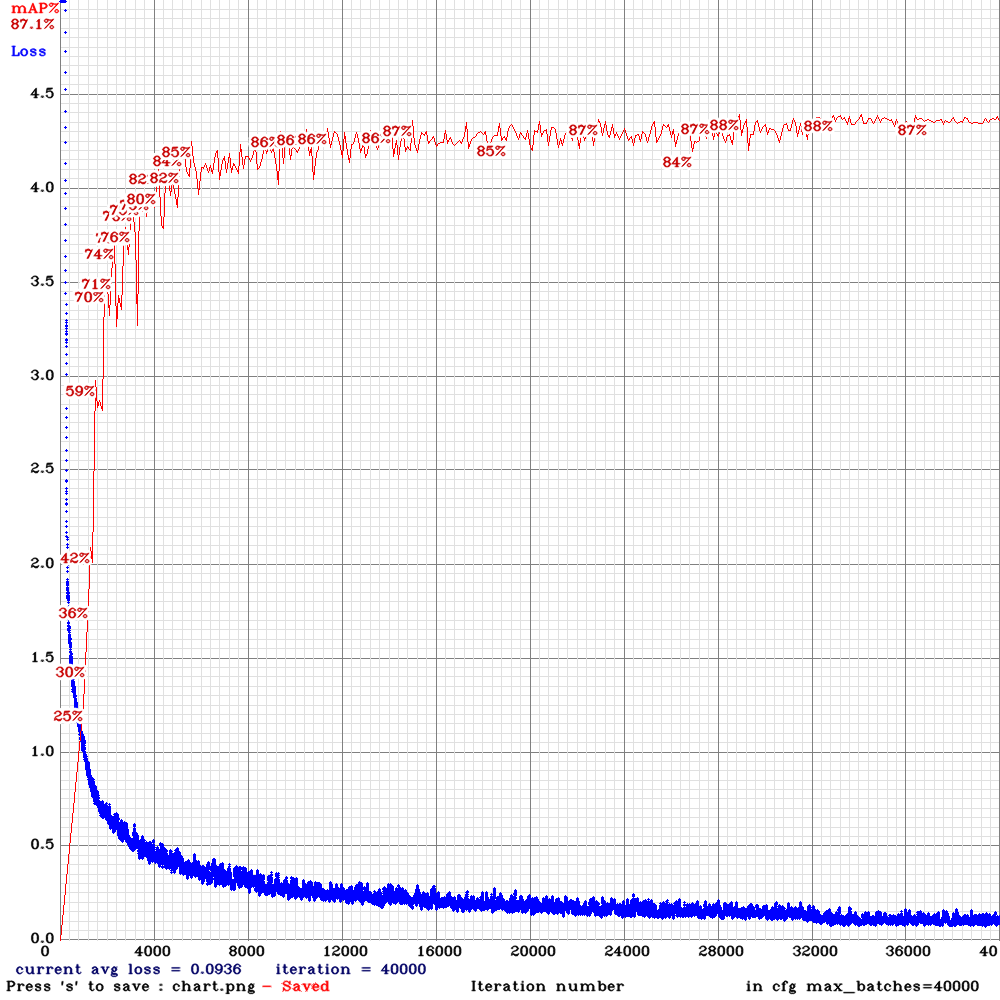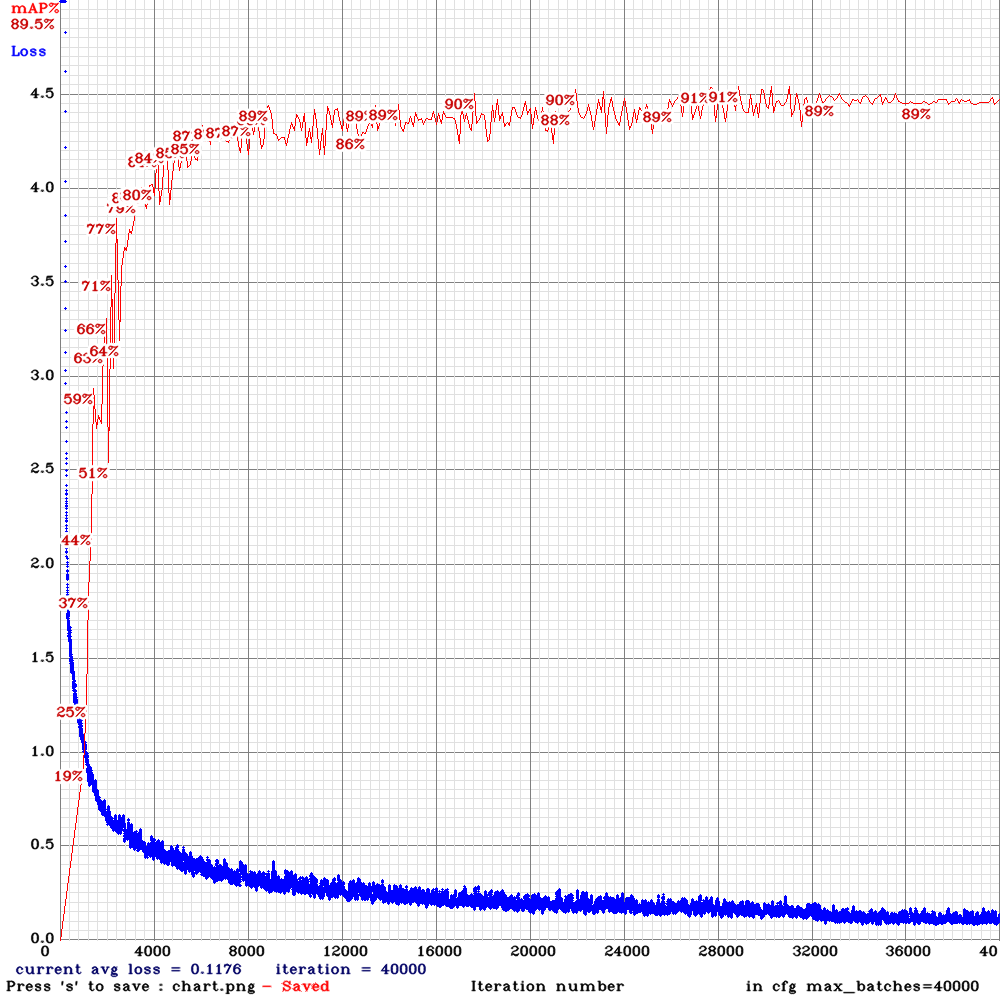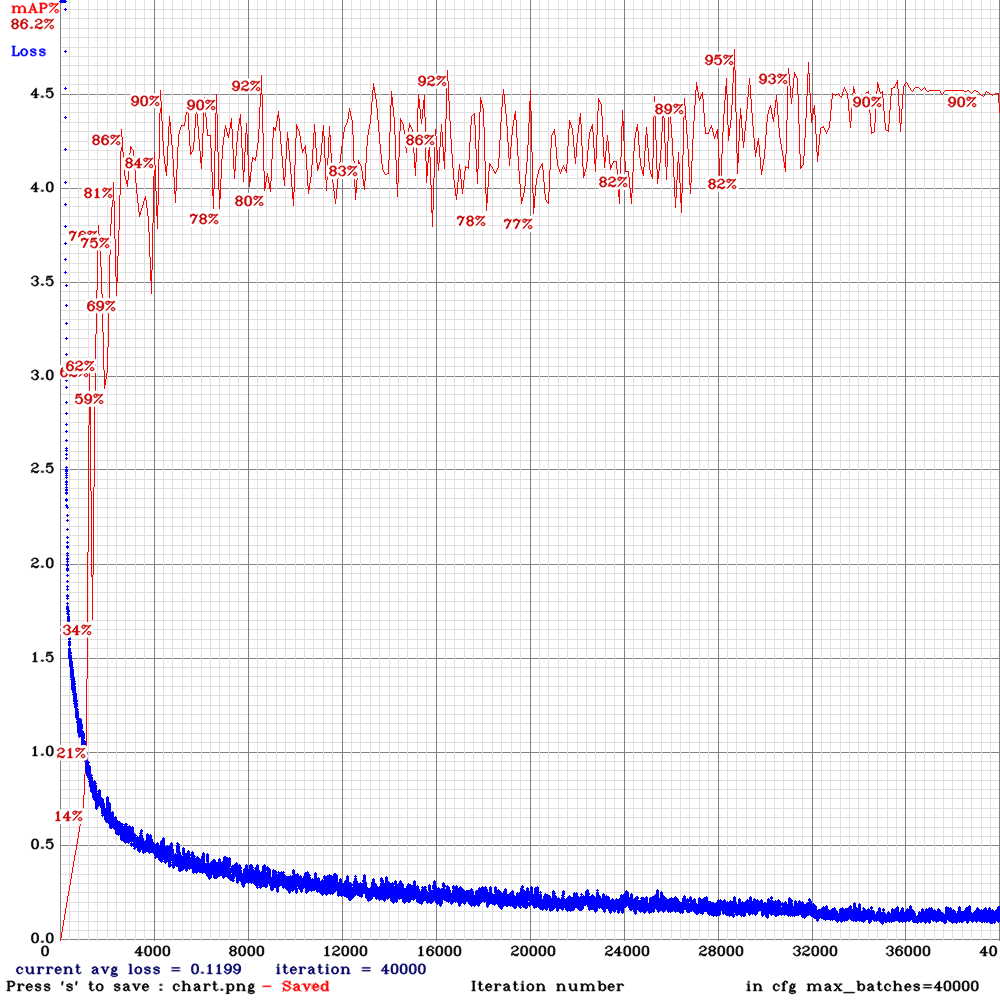The only thing that surprised me was how similar the charts were within each set of max_batches. So much so, that I used GIMP to combine several of them together to better compare the results.

For example, when I combine into a single image the 50%, 70%, 85% and 99% charts from max_batches=20000, the result looks like this: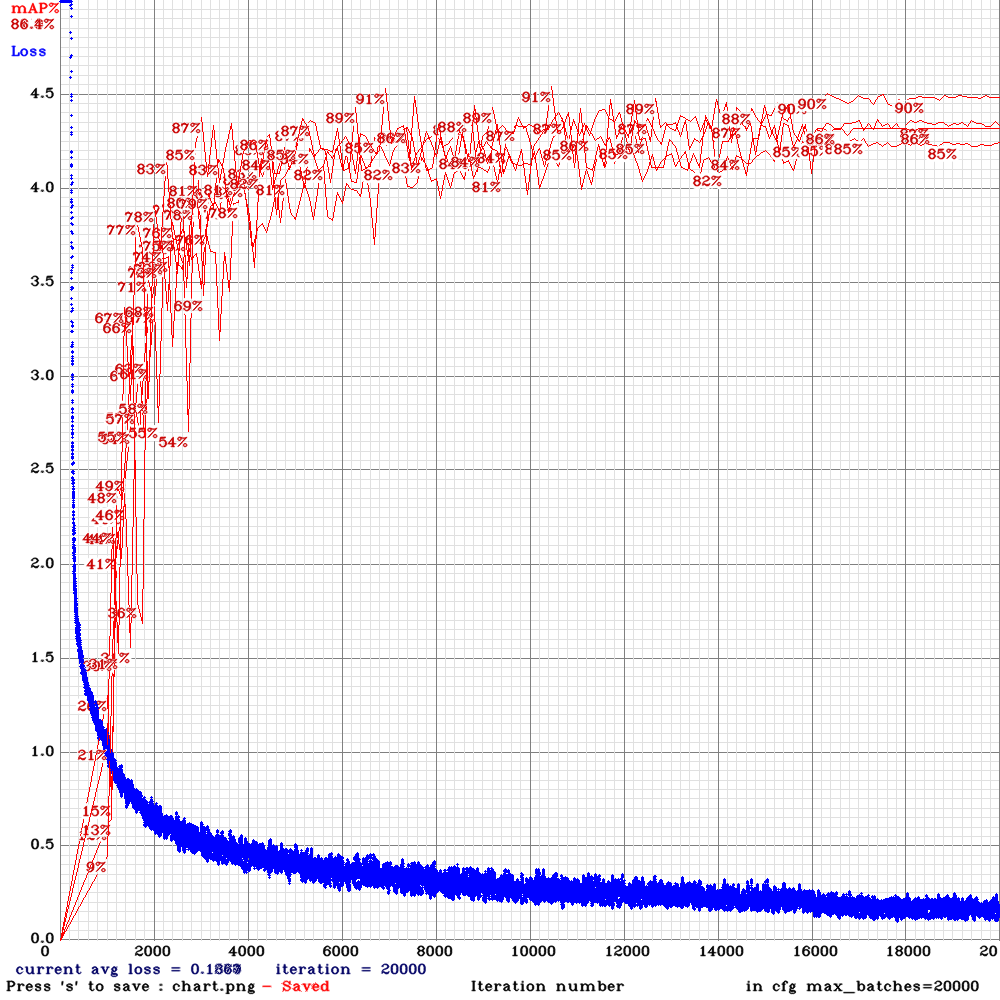As the max_batches increases, the loss is progressively smaller, which is the expected behaviour. And the training/validation ratio has little impact on either the loss or the mAP (mean average precision) once you have enough marked images combined with a large value for max_batches. Only at the low end when max_batches is too small does the training/validation ratio impact the results, but we already know from the results above that those neural networks are unusable/inconsistent.

## Predictions

The following shows the final results of various images with the ANN when max_batches is set to 10K and 40K. The most significant difference is the probability in many of these images:

max_batches=10000 max_batches=40000 max_batches=10000 max_batches=40000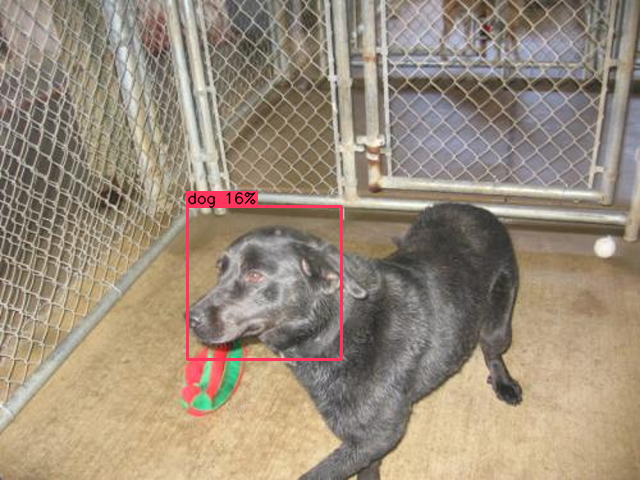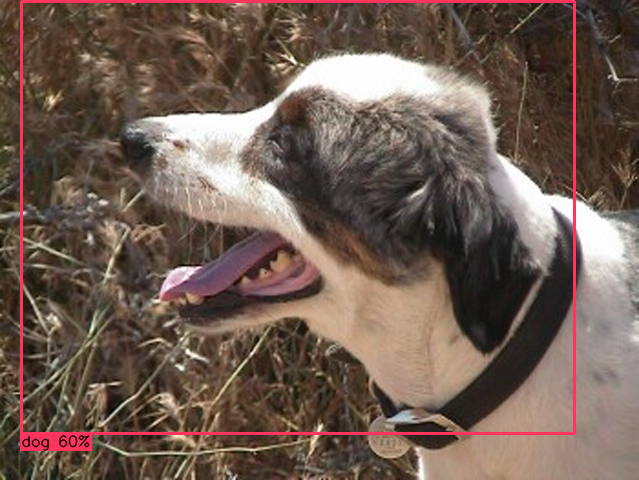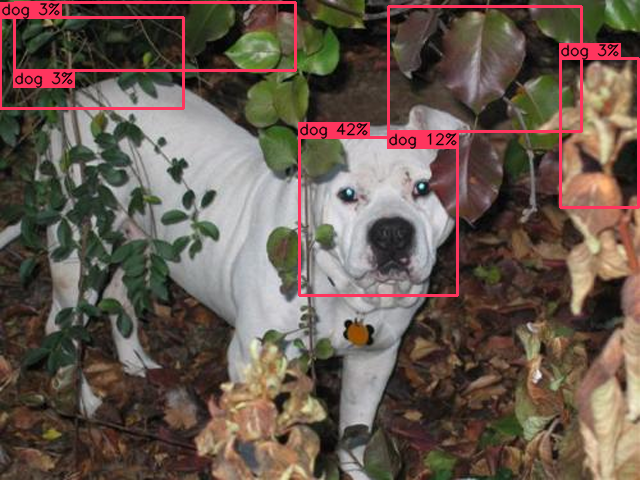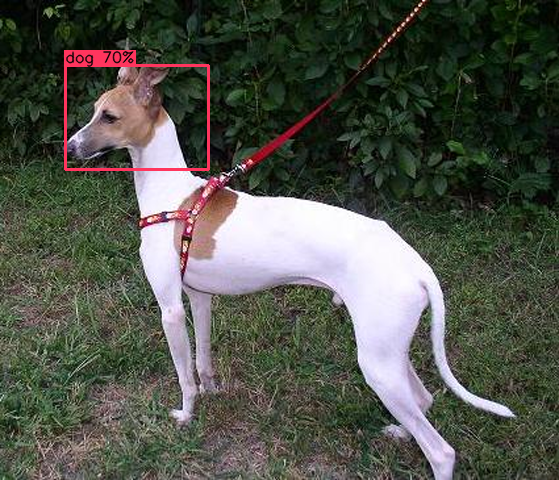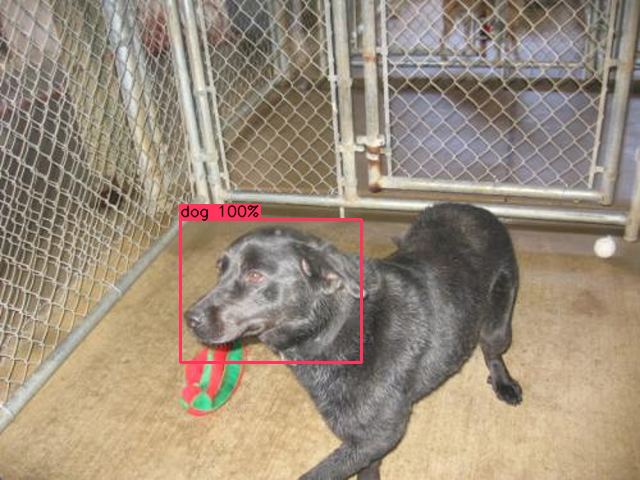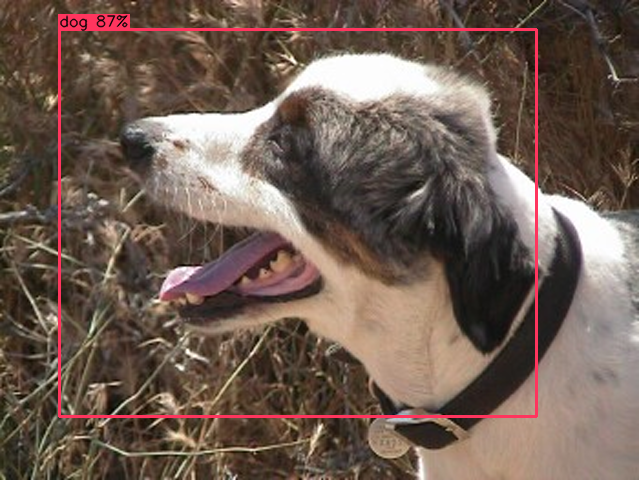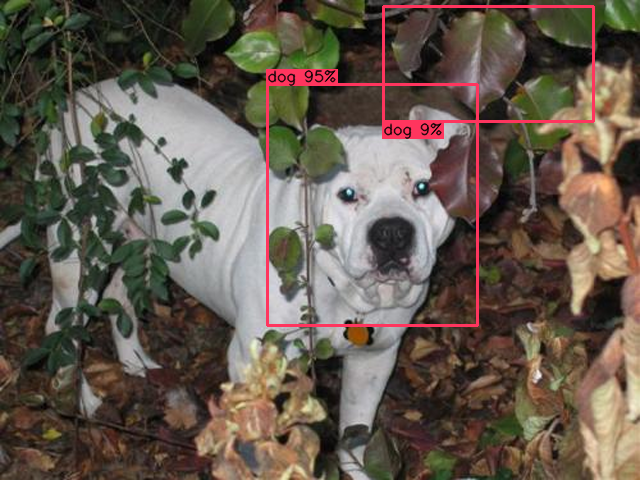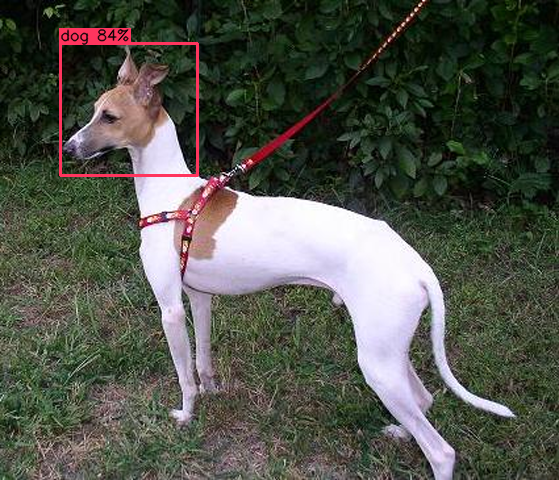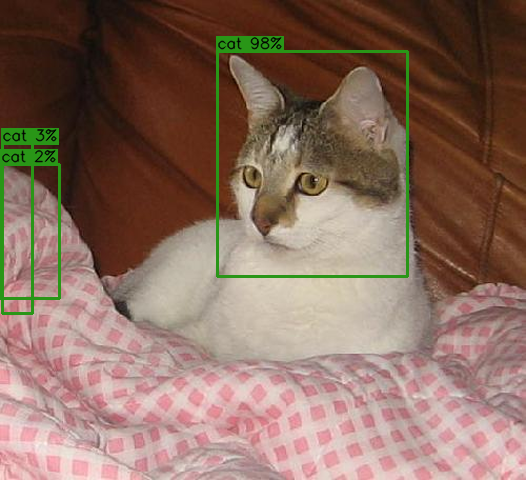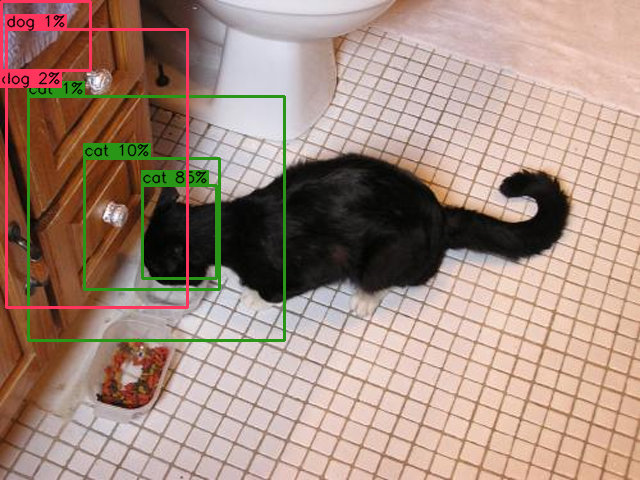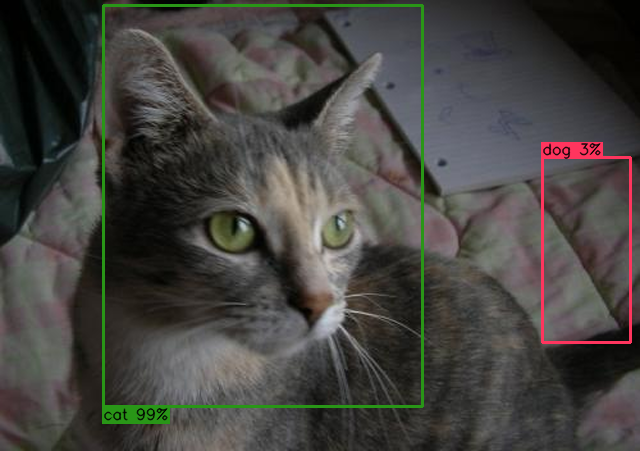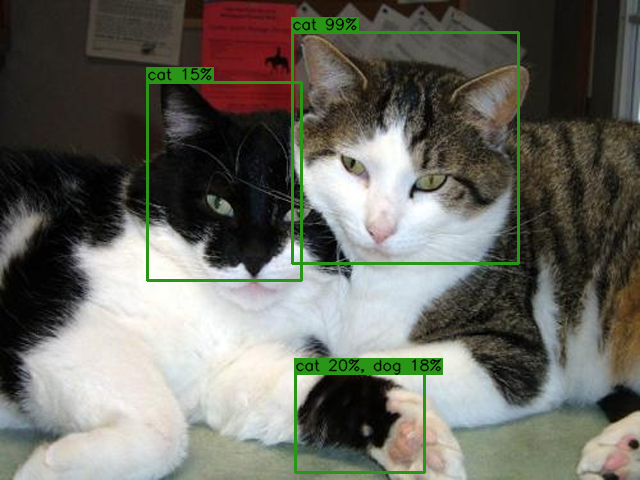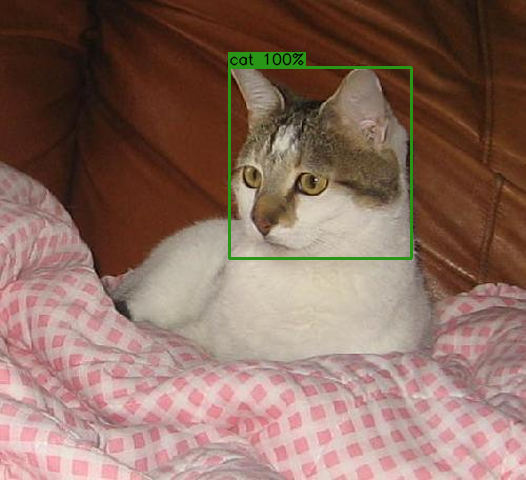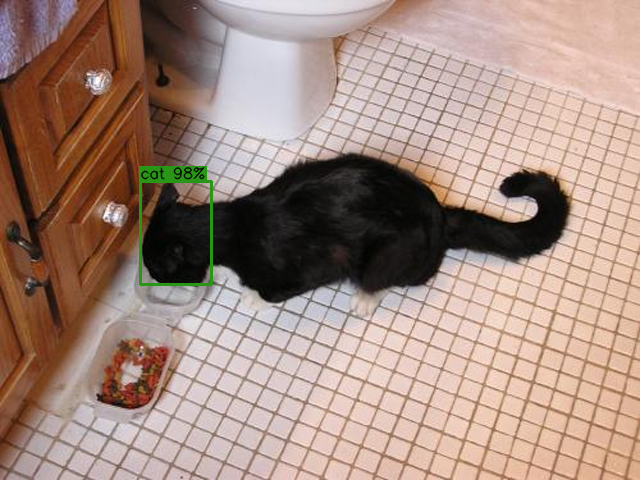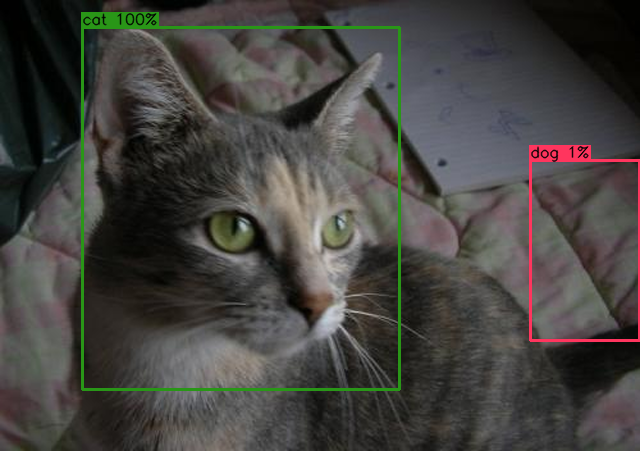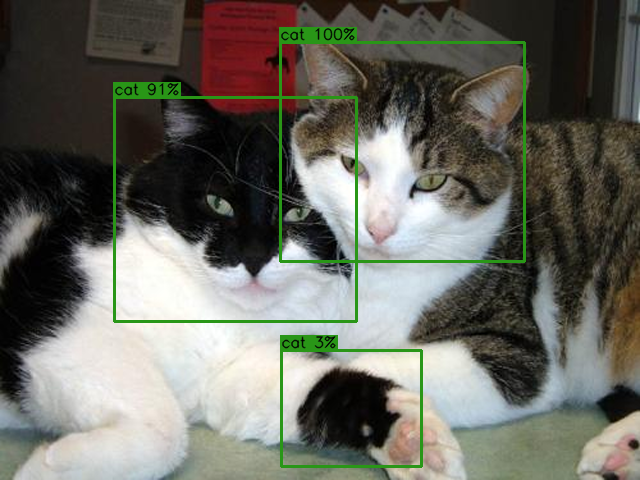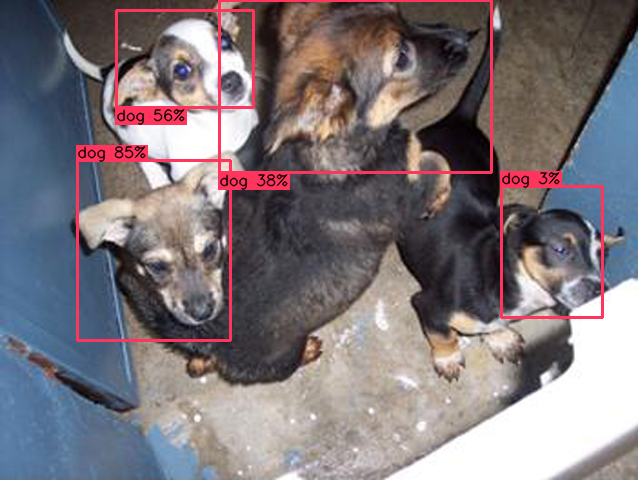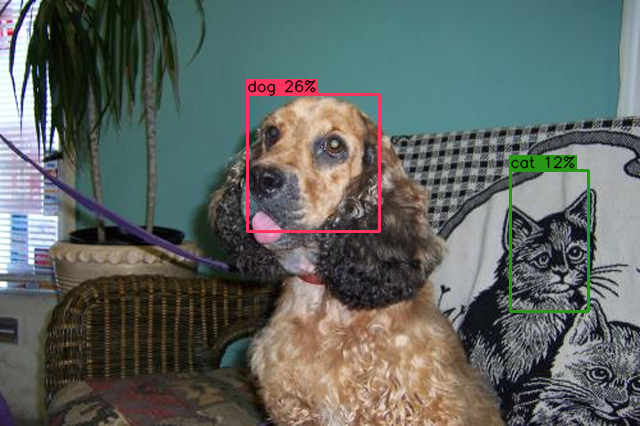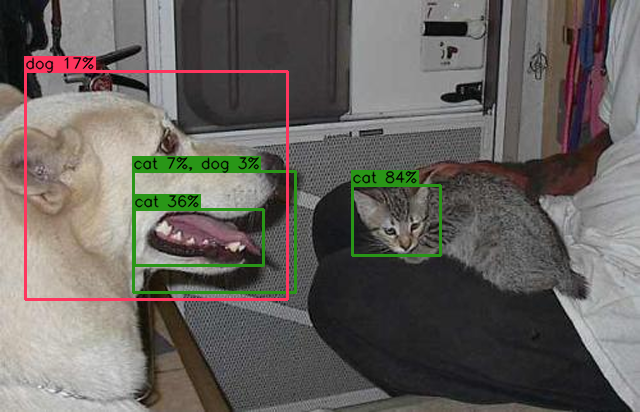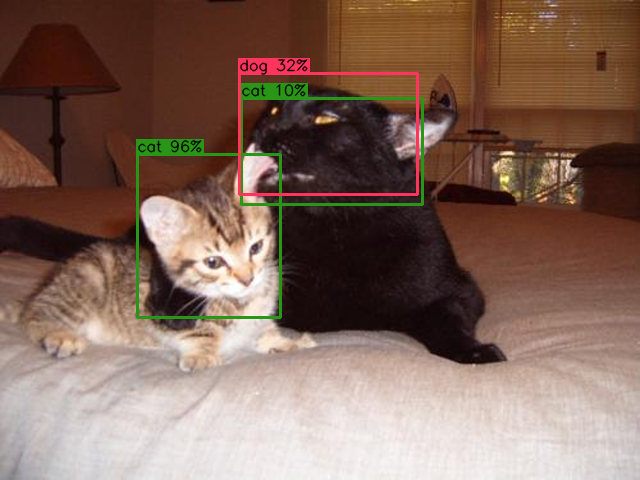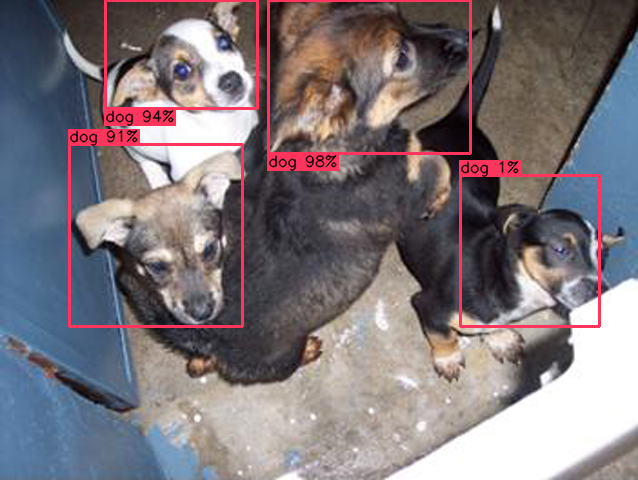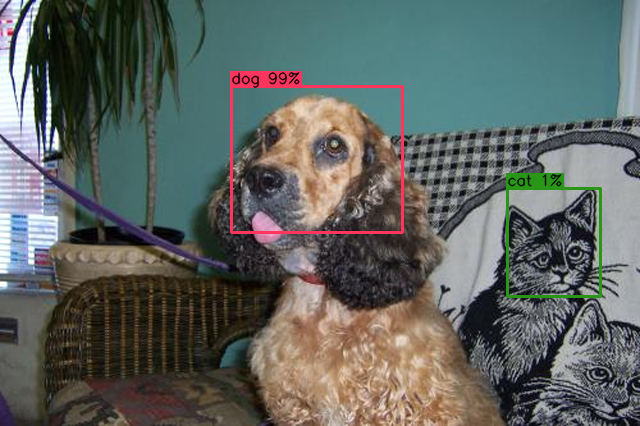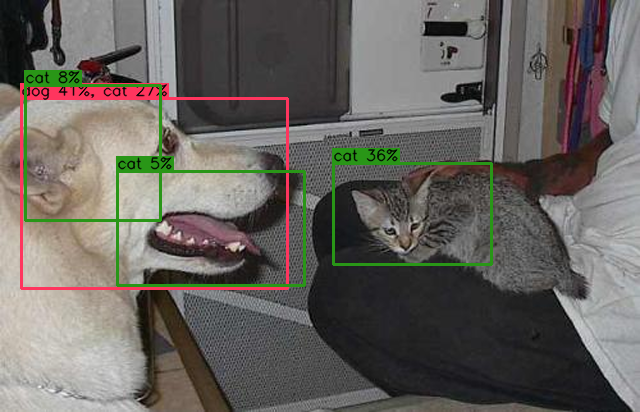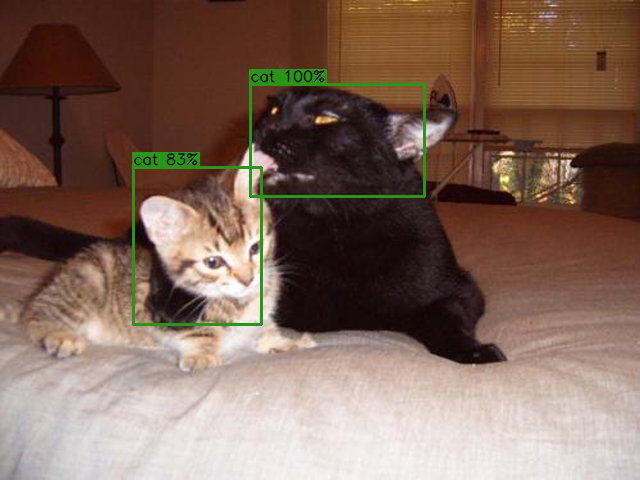In reality, when applying an ANN to images in a commercial application, you'd never use a threshold as low as 1%, so some of those images with multiple predictions would actually show up with a simpler region of interest. Thus, images like this one:...becomes this: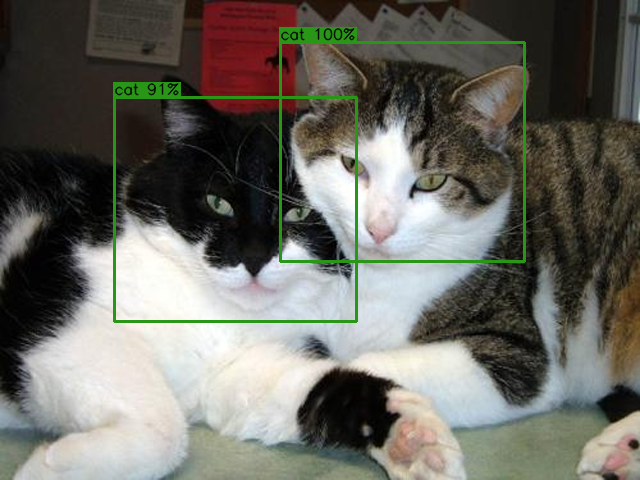## Image Rotation

The last item worth mentionning is the impact of the image's rotation on the effectiveness of the ANN. If the neural network needs to detect it, then it must be trained with it! A visual demonstration:

 original image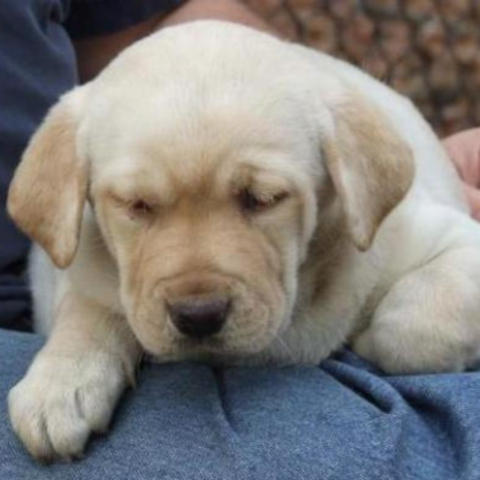rotation=0°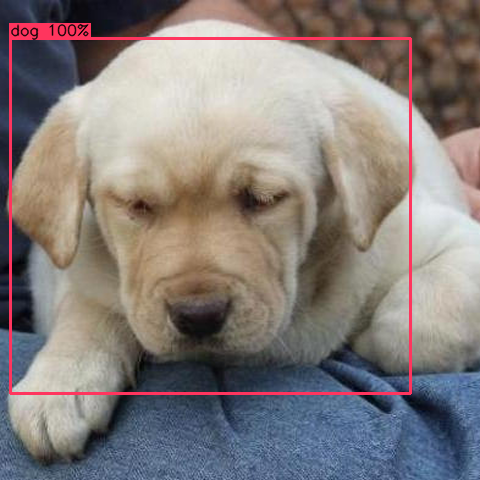rotation=45°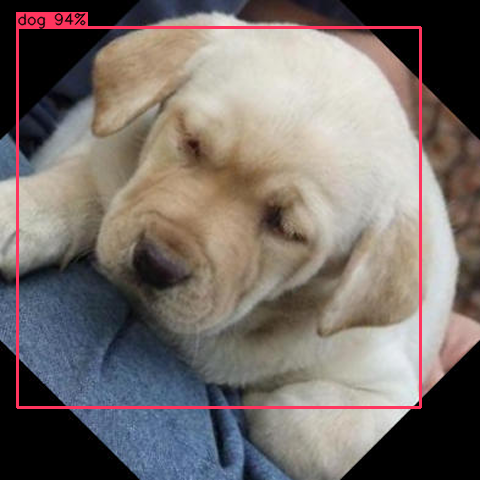rotation=90°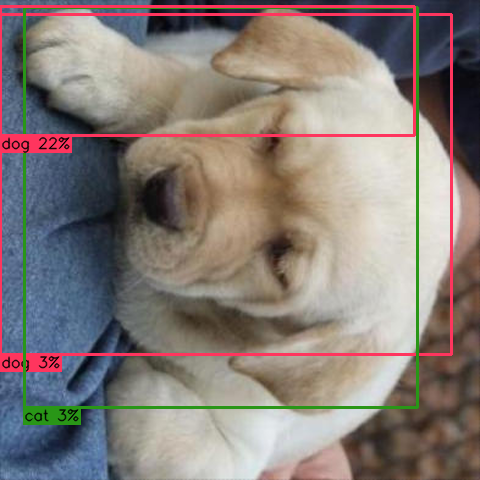rotation=135°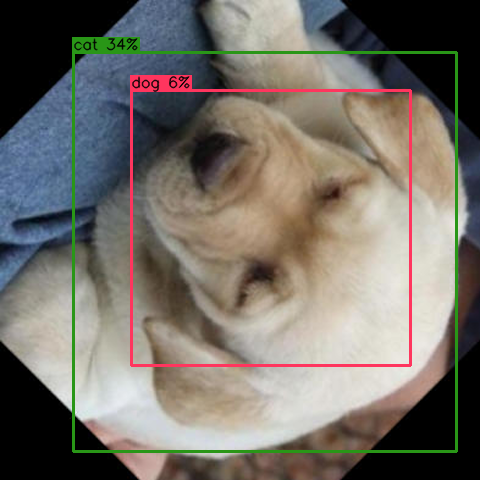rotation=180°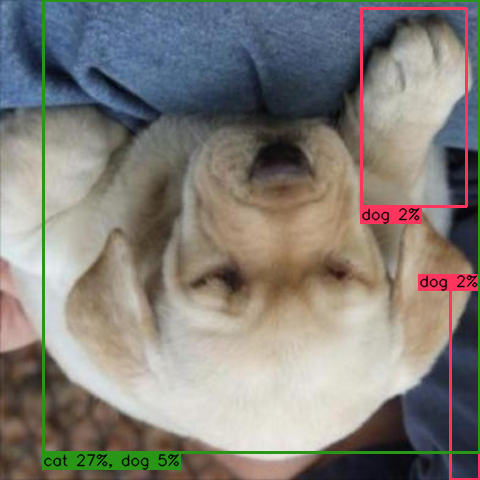rotation=225°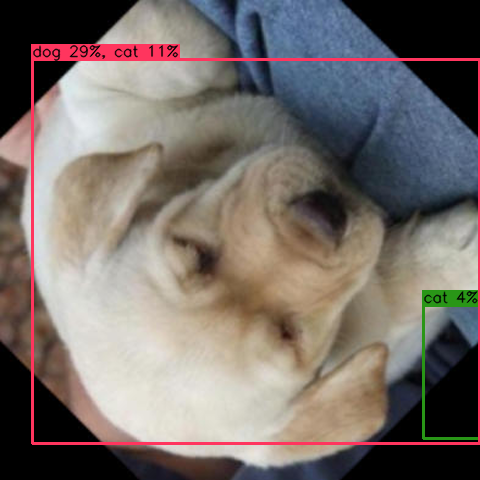rotation=270°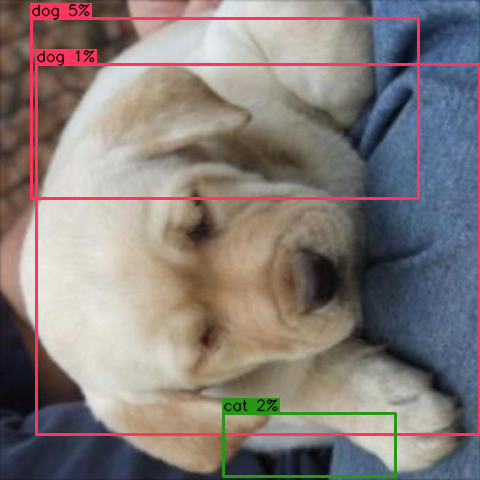rotation=315°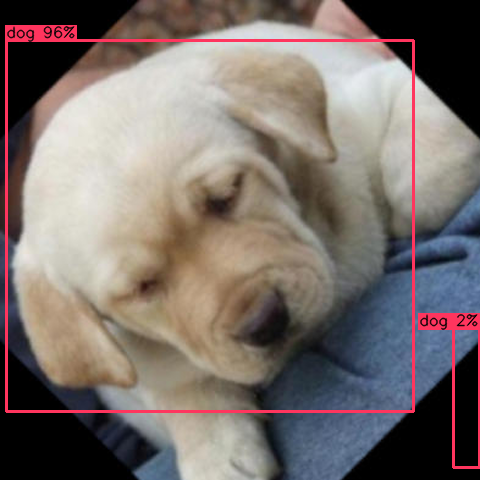The original image was correctly identified with 100% certainty as a dog. By the time the image is rotated upside down, the network is now predicting the image is a cat instead of a dog.

As of 2020-01-04, Darknet's data augmentation doesn't yet support rotation. There is a "angle=..." entry in the YOLO configuration files, but AlexeyAB has commented that it is only available for classification, not detector.